US5050974A - Optical system for endoscopes - Google Patents

Info

Publication number
US5050974A
US5050974A US07/406,383 US40638389A US5050974A US 5050974 A US5050974 A US 5050974A US 40638389 A US40638389 A US 40638389A US 5050974 A US5050974 A US 5050974A
Authority
US
United States
Prior art keywords
sub
optical system
lens component
ν
endoscopes
Prior art date
Legal status (The legal status is an assumption and is not a legal conclusion. Google has not performed a legal analysis and makes no representation as to the accuracy of the status listed.)
Application number
US07/406,383
Inventor
Yoshiharu Takasugi
Hiroyuki Fukuda
Current Assignee (The listed assignees may be inaccurate. Google has not performed a legal analysis and makes no representation or warranty as to the accuracy of the list.)
Olympus Corp
Original Assignee
Olympus Corp
Priority date (The priority date is an assumption and is not a legal conclusion. Google has not performed a legal analysis and makes no representation as to the accuracy of the date listed.)
Filing date
Publication date
Priority to JP63226531A priority Critical patent/JP2596810B2/en
Priority to JP63-226531 priority
Application filed by Olympus Corp filed Critical Olympus Corp
Assigned to OLYMPUS OPTICAL CO., LTD. reassignment OLYMPUS OPTICAL CO., LTD. ASSIGNMENT OF ASSIGNORS INTEREST. Assignors: FUKUDA, HIROYUKI, TAKASUGI, YOSHIHARU
Application granted granted Critical
Publication of US5050974A publication Critical patent/US5050974A/en
Anticipated expiration legal-status Critical
Application status is Expired - Lifetime legal-status Critical

• 206010010071 Coma Diseases 0 description 3
• 230000004075 alteration Effects 0 description 12
• 201000009310 astigmatism Diseases 0 description 2
• 230000000875 corresponding Effects 0 description 1
• 230000002708 enhancing Effects 0 description 1
• 239000000835 fiber Substances 0 description 1
• 238000003384 imaging method Methods 0 abstract claims description 22
• 238000000034 methods Methods 0 description 1
• 239000000203 mixtures Substances 0 description 7
• 238000009740 moulding (composite fabrication) Methods 0 description 2
• 230000003287 optical Effects 0 abstract claims description title 134
• 230000003405 preventing Effects 0 description 2
• 230000035945 sensitivity Effects 0 description 1
• 238000007493 shaping process Methods 0 description 1
• 238000004904 shortening Methods 0 description 1
• 230000003313 weakening Effects 0 description 4

Images

•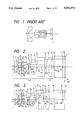•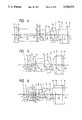•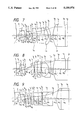•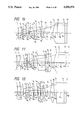•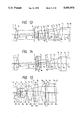•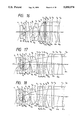•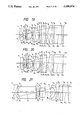•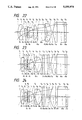•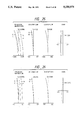•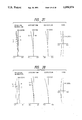•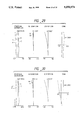••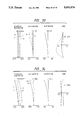•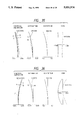•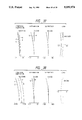•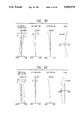•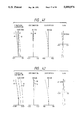•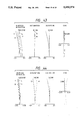•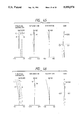•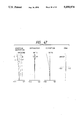Classifications

• AHUMAN NECESSITIES
• A61MEDICAL OR VETERINARY SCIENCE; HYGIENE
• A61BDIAGNOSIS; SURGERY; IDENTIFICATION
• A61B1/00Instruments for performing medical examinations of the interior of cavities or tubes of the body by visual or photographical inspection, e.g. endoscopes; Illuminating arrangements therefor
• A61B1/04Instruments for performing medical examinations of the interior of cavities or tubes of the body by visual or photographical inspection, e.g. endoscopes; Illuminating arrangements therefor combined with photographic or television appliances
• BPERFORMING OPERATIONS; TRANSPORTING
• B29WORKING OF PLASTICS; WORKING OF SUBSTANCES IN A PLASTIC STATE IN GENERAL
• B29DPRODUCING PARTICULAR ARTICLES FROM PLASTICS OR FROM SUBSTANCES IN A PLASTIC STATE
• B29D11/00Producing optical elements, e.g. lenses or prisms
• GPHYSICS
• G02OPTICS
• G02BOPTICAL ELEMENTS, SYSTEMS, OR APPARATUS
• G02B17/00Systems with reflecting surfaces, with or without refracting elements
• GPHYSICS
• G02OPTICS
• G02BOPTICAL ELEMENTS, SYSTEMS, OR APPARATUS
• G02B21/00Microscopes
• G02B21/02Objectives
• GPHYSICS
• G02OPTICS
• G02BOPTICAL ELEMENTS, SYSTEMS, OR APPARATUS
• G02B23/00Telescopes, e.g. binoculars; Periscopes; Instruments for viewing the inside of hollow bodies; Viewfinders; Optical aiming or sighting devices
• G02B23/24Instruments or systems for viewing the inside of hollow bodies, e.g. fibrescopes
• G02B23/26Instruments or systems for viewing the inside of hollow bodies, e.g. fibrescopes using light guides
• GPHYSICS
• G02OPTICS
• G02BOPTICAL ELEMENTS, SYSTEMS, OR APPARATUS
• G02B9/00Optical objectives characterised both by the number of the components and their arrangements according to their sign, i.e. + or -
• G02B9/60Optical objectives characterised both by the number of the components and their arrangements according to their sign, i.e. + or - having five components only
• GPHYSICS
• G02OPTICS
• G02BOPTICAL ELEMENTS, SYSTEMS, OR APPARATUS
• G02B9/00Optical objectives characterised both by the number of the components and their arrangements according to their sign, i.e. + or -
• G02B9/62Optical objectives characterised both by the number of the components and their arrangements according to their sign, i.e. + or - having six components only

Abstract

An optical system for endoscopes so adapted as to be usable with video endoscopes, comprising an imaging lens system equipped with an aperture stop and a field lens component arranged on the image side thereof, and so designed as to prevent the color shading from being produced by selecting an adequate focal length for the field lens component.

Description

BACKGROUND OF THE INVENTION

a) Field of the Invention

The present invention relates to an imaging optical system for endoscopes.

b) Description of the Prior Art

Fiberscopes using image guide fiber bundles are practically employed in a large number as endoscopes. In the recent days, however, video endoscopes using various types of solid-state image pickup devices in place of the image guides are also used in a large number.

The video color imaging systems are classified roughly into the field sequential color system and the dot sequential color systems. The latter has a composition comprising a color encoding filter which is composed of mini-size color filters integrated in mosaic patterns corresponding to the picture elements of a solid-state image pickup device (usually referred to as the color mosaic filter). When a wide space is reserved between the filter and the light-receiving surface of the solid-state image pickup device, however, the light incident on the solid-stage image pickup device does not fall on the picture element on which the light is originally to fall after passing through the color encoding filter but on the neighboring picture element, thereby producing color ununiformity on an image (hereinafter referred to as the color shading). The color shading produced in this way cannot be avoided in an optical system of the same type as the optical system for endoscopes disclosed by Japanese Unexamined Published Patent Application No. 173415/62 wherein the principal ray l falls obliquely on an image pickup device I as shown in FIG. 1.

SUMMARY OF THE INVENTION

A primary object of the present invention is to provide an imaging optical system for endoscopes capable of forming an image free from the color shading even when the solid-state image pickup devices comprising the mosaic filters are used.

The optical system for endoscopes according to the present invention has the fundamental composition illustrated in FIG. 2 wherein a field lens component LF is arranged on the image side of an imaging lens system LM comprising an aperture stop S and a solid-state image pickup device is arranged on the side where rays emerge from said field lens component LF. Further, the optical system for endoscopes according to the present invention is so designed as to satisfy the following condition (1):

f.sub.F <10f                                               (1)

wherein the reference symbol fF represents focal length of the field lens component LF and the reference symbol f designates focal length of the optical system as a whole.

By selecting the composition described above, the optical system according to the present invention is so adapted as to allow the principal ray to be incident nearly perpendicularly on the image surface thereof and therefore capable of preventing the color shading from being produced.

If the above-mentioned field lens component LF has too long a focal length, the optical system will have too long a total length and too large an outside diameter, thereby being unsuited for use with endoscopes. In order to make the optical system compact as a whole, it is desirable to design the optical system so as to satisfy the above-mentioned condition (1). If the condition (1) is not satisfied, the optical system will undesirably have too long a total length and too large an outside diameter.

The optical system for endoscopes according to the present invention comprises the field lens component LF arranged on the image side of the imaging lens system LM comprising the aperture stop and is so designed as to satisfy the condition (1). It is desirable to use, as at least one of the surfaces of the field lens component or in the vicinity thereof, an aspherical surface including portions whose refractive functions are gradually weakened as the portions are farther from the optical axis toward the margin.

The optical system according to the present invention is so adapted as to prevent the color shading by arranging the field lens component LF on the image side of the imaging lens system LM so that the principal ray is incident perpendicularly on the image surface thereof. In an ordinary optical system composed only of spherical lenses wherein rays are incident on the image surface thereof at different angles depending on image heights, it is desirable to arrange an aspherical surface in the vicinity of the field lens component so that angles of incidence of the rays are not different on the image surface.

When it is assumed that the rays are perpendicularly incident on the image surface at all the image heights in the optical system according to the present invention, tracing of the rays at all the image heights in the reverse direction toward the object side along the optical axis will clarify that all the rays pass through the center of the aperture stop. In other words, no spherical aberration is produced by the rays travelling from the image surface toward the object side along the optical axis in the telecentric optical system arranged on the image side of the aperture stop. Accordingly, it is desirable to arrange an aspherical surface at a location where spherical aberration is produced remarkably when tracing is made in the reverse direction from the image side, i.e., at a location in the vicinity of the field lens component LF.

An optical system for endoscopes is generally designed as the retrofocus type consists of a front lens unit which has negative refractive power as a whole and is arranged on the object side of an aperture stop, and a rear lens unit which has positive refractive power as a whole and is arranged on the image side of the aperture stop. Normally, a positive lens component produces negative spherical aberration since it refracts rays more largely as the portions thereof become farther from the optical axis. It is therefore desirable to design the aspherical surface to be arranged in the vicinity of the field lens component LF so as to include portions whose refractive functions are gradually weakened as they are farther from the optical axis toward the margin.

The weakening of refractive function of a positive lens component means weakening of the converging function, i.e., the positive power thereof, whereas weakening of refractive function of a negative lens component means weakening of the diverging function, i.e., the negative power thereof.

When the optical system for endoscopes according to the present invention has a large field angle of approximately 100° or wider, barrel-shaped distortion is produced remarkably as image height increases. In order to correct this distortion, it is desirable to arrange, on the object side of the aperture stop, an aspherical surface having refractive function which is gradually weakened as the surface portions are farther from the optical axis toward the margin.

In order to correct distortion, it is sufficient to arrange an aspherical surface at a location at which the principal ray is high. For the above-described aspherical surface to be arranged in the vicinity of the field lens component LF in the optical system for endoscopes according to the present invention, however, a shape must be selected in such a manner that the rays at all the image heights are incident on an image pickup device at a definite angle (perpendicularly). It is therefore desirable to correct distortion by arranging the aspherical surface at a location at which rays are high on the object side of the aperture stop. The aspherical surface to be arranged for correcting distortion should include such portions as to gradually weaken the refractive function of the lens component as the portions are farther from the optical axis toward the margin.

The lens component which is located on the object side of the aperture stop and at which ray is the highest is the first lens component, and this lens component is usually designed as a negative lens component in an optical system for endoscopes. Remarkable distortion is produced by the object side surface of the first lens component (the first surface of the optical system) since said surface refracts rays outward more largely as the surface portions are farther from the optical axis toward the margin. When the first surface is designed as an aspherical surface including portions which weaken the refractive function of the lens component as the portions are farther from the optical axis toward the margin, for example, it is possible to correct distortion owing to the fact that the aspherical surface moderates refraction of the rays.

When only one aspherical surface is arranged on each of the object side and image side of the aperture stop, an attempt to correct distortion while keeping a definite angle of incidence on the image pickup device will produce astigmatism. Correction of this astigmatism will diversify the angle of incidence on the image pickup device, thereby making it impossible to correct aberrations sufficiently. For correcting the aberrations, it is desirable to use an additional aspherical surface.

Then, it is desirable to design the optical system for endoscopes according to the present invention so as to satisfy the following condition (2):

D.sub.1 >0.2f                                              (2)

wherein the reference symbol D1 represents the optical path length to be reserved between the aperture stop S and the field lens component LF.

The solid-state image pickup device has sensitivity for the light having infrared wavelength and degrades color reproducibility on an image. In order to correct this defect, it is usually necessary to arrange an optical filter such as an infrared cut filter or the similar member in the optical path of the optical system. The condition (2) is required for arranging the optical member such as an optical filter. In order to arrange an optical filter having the desired characteristic, D1 should desirably satisfy the condition (2). If the condition (2) is not satisfied, it will be difficult to arrange an optical filter in the optical system for endoscopes according to the present invention.

It is further desirable to design the optical system for endoscopes according to the present invention so as to satisfy the following condition (3):

0.2f<D.sub.2 <5f                                           (3)

wherein the reference symbol D2 represents optical path length as measured from the field lens component LF to the imaging surface, which should be measured from the vertex of the object side surface of the field lens component LF to the imaging surface when the field lens component LF is integrated with an image pickup device, or from the vertex of the image side surface of the field lens component LF to the imaging surface when the field lens component LF is separate from an image pickup device.

When D2 has too small a value, it will be impossible to reserve a space for arranging, for example, an optical low pass filter for correcting moire. If D2 has too large a value, in contrast, it will be possible to reserve a space sufficient for arranging the optical low pass filter or the similar member, but the optical system will have too long a total length and cannot be compact.

When a plane parallel plate such as an optical filter is arranged on the object side of the field lens component LF, i.e., in the imaging lens system, or between the imaging lens system and the field lens component LF in the optical system according to the present invention, the optical system is divided into a front subsystem located on the object side of the plane parallel plate and a rear subsystem located on the image side of the plane parallel plate. When two or more plane parallel plates are arranged in such a case, the optical system is to be divided into the front subsystem and the rear subsystem taking the plane parallel plate closest to the field lens component LF as a boundary. The front subsystem may be composed of a plural number of lens components including positive lens component(s) and negative lens component(s) for correcting aberrations. When the front subsystem has the above-described composition, it is desirable to design the subsystem so as to satisfy the following conditions (4), (5), and (6):

f.sub.I >0.3f                                              (4)

|f.sub.n |<4f                            (5)

0.3f<f.sub.p <5f                                           (6)

wherein the reference symbol f1 represents focal length of the front subsystem, the reference symbol fn designates focal length of the negative lens components (not including the negative lens elements of the cemented doublets) and the reference symbol fp denote total focal length of the lens elements not including the negative lens element(s) arranged in the front subsystem.

The condition (4) defines focal length of the front subsystem. As f1 becomes shorter, the optical path length is shorter between the front subsystem and the rear subsystem. If the condition (4) is not satisfied, it will therefore be impossible to arrange an optical filter.

If fn or fp exceeds the upper limit of the condition (5) or condition (6), the optical system will have too long a total length and too large an outside diameter, thereby making the optical system unsuited for use with endoscopes. If fp has a value smaller than the lower limit of the condition (6), in contrast, there will be available no space for arranging an optical filter.

BRIEF DESCRIPTION OF THE DRAWINGS

FIG. 1 shows a sectional view illustrating the conventional optical system for endoscopes;

FIG. 2 through FIG. 24 show sectional views illustrating Embodiments 1 through 23 of the optical system for endoscopes according to the present invention;

FIG. 25 through FIG. 47 show curves illustrating aberration characteristics of the Embodiments 1 through 23 of the present invention.

DETAILED DESCRIPTION OF THE PREFERRED EMBODIMENTS

Now, the present invention will be described more detailedly with reference to the accompanying drawings and the numerical data given below in the form of numerical data:

EMBODIMENT 1

______________________________________f =      1.000,    F/3.941, 2ω = 133.698°IH =     1.0049______________________________________r.sub.1 = ∞      d.sub.1 = 0.3045                  n.sub.1 = 1.88300                             ν.sub.1 = 40.78r.sub.2 = 0.5725      d.sub.2 = 0.2253r.sub.3 = 2.9842      d.sub.3 = 0.3228                  n.sub.2 = 1.84666                             ν.sub.2 = 23.78r.sub.4 = -1.1924      d.sub.4 = 0.0609r.sub.5 = ∞ (stop)      d.sub.5 = 0.0609r.sub.6 = -1.2314      d.sub.6 = 0.1827                  n.sub.3 = 1.80518                             ν.sub.3 = 25.43r.sub.7 = 1.6809      d.sub.7 = 0.6395                  n.sub.4 = 1.51633                             ν.sub.4 = 64.15r.sub.8 = -0.7893      d.sub.8 = 0.0609r.sub.9 =  5.5341      d.sub.9 = 0.1583                  n.sub.5 = 1.84666                             ν.sub.5 = 23.78r.sub.10 = 1.7381      d.sub.10 = 0.8222                  n.sub.6 = 1.51633                             ν.sub.6 = 64.15r.sub.11 = -1.7381      d.sub.11 = 0.1279r.sub.12 = ∞      d.sub.12 = 0.9135                  n.sub.7 = 1.52000                             ν.sub.7 = 74.00r.sub.13 = ∞      d.sub.13 = 0.2436r.sub.14 = 2.2400      d.sub.14 = 0.7004                  n.sub.8 = 1.51633                             ν.sub.8 = 64.15r.sub.15 = 19.9239      d.sub.15 = 0.3045r.sub.16 = ∞      d.sub.16 = 1.1389                  n.sub.9 = 1.54869                             ν.sub.9 = 45.55r.sub.17 = ∞      d.sub.17 = 0.2436                  n.sub.10 = 1.51633                             ν.sub.10 = 64.15r.sub.18 =  ∞______________________________________f.sub.F =  4.823,    D.sub.1 =                    0.972,   D.sub.2 =                                   1.2f.sub.I =  1.481,    f.sub.n =                    -0.648,  f.sub.p =                                   1.314______________________________________
EMBODIMENT 2

______________________________________f =      1.000,     F/4.815, 2ω = 133°IH =     0.9857______________________________________r.sub.1 = ∞      d.sub.1 = 0.2987                  n.sub.1 = 1.88300                             ν.sub.1 = 40.78r.sub.2 = 0.4920      d.sub.2 = 0.2210r.sub.3 = 30.2837      d.sub.3 = 0.2747                  n.sub.2 = 1.84666                             ν.sub.2 = 23.78r.sub.4 = -1.1561      d.sub.4 = 0.0597r.sub.5 = ∞ (stop)      d.sub.5 = 0.1016r.sub.6 = -4.1194      d.sub.6 = 0.1792                  n.sub.3 = 1.80518                             ν.sub.3 = 25.43r.sub.7 = 2.1470      d.sub.7 = 0.4779                  n.sub.4 = 1.51633                             ν.sub.4 = 64.15r.sub.8 = -0.7172      d.sub.8 = 0.1135r.sub.9 =  -46.5690      d.sub.9 = 0.1553                  n.sub.5 = 1.84666                             ν.sub.5 = 23.78r.sub.10 = 1.2640      d.sub.10 = 0.7168                  n.sub.6 = 1.51633                             ν.sub.6 = 64.15r.sub.11 = -1.7141      d.sub.11 = 0.1254r.sub.12 = ∞      d.sub.12 = 0.2389                  n.sub.7 = 1.51633                             ν.sub.7 = 64.15r.sub.13 = ∞      d.sub.13 = 0.4182                  n.sub.8 = 1.52000                             ν.sub.8 = 74.00r.sub.14 = ∞      d.sub.14 = 0.2389                  n.sub.9 = 1.51633                             ν.sub.9 = 64.15r.sub.15 = ∞      d.sub.15 = 0.0896r.sub.16 = 2.3145      d.sub.16 = 0.7168                  n.sub.10 = 1.53172                             ν.sub.10 = 48.90r.sub.17 = -8.4075      d.sub.17 = 0.6094r.sub. 18 = ∞      d.sub.18 = 1.1171                  n.sub.11 = 1.54869                             ν.sub.11 = 45.55r.sub.19 = ∞      d.sub.19 = 0.2389                  n.sub.12 = 1.51633                             ν.sub.12 = 64.15r.sub.20 = ∞______________________________________f.sub.F =  3.494,    D.sub.1 =                    0.805,   D.sub.2 =                                   1.488f.sub.I =  1.954,    f.sub.n =                    -0.557,  f.sub.p =                                   1.18______________________________________
EMBODIMENT 3

______________________________________f =      1.000,    F/5.884, 2ω = 96.902°IH =     0.8238______________________________________r.sub.1 = ∞      d.sub.1 = 0.2496                  n.sub.1 = 1.88300                             ν.sub.1 = 40.78r.sub.2 = 1.1373      d.sub.2 = 0.3145r.sub.3 = ∞      d.sub.3 = 2.5062                  n.sub.2 = 1.80610                             ν.sub.2 = 40.95r.sub.4 = ∞ (stop)      d.sub.4 = 0.0999r.sub.5 = 2.2247      d.sub.5 = 0.3645                  n.sub.3 = 1.72916                             ν.sub.3 = 54.68r.sub.6 = -2.2247      d.sub.6 = 0.0999r.sub.7 = ∞      d.sub.7 = 0.7489                  n.sub.4 = 1.52000                             ν.sub.4 = 74.00r.sub.8 = ∞      d.sub.8 = 0.6990r.sub.9  = ∞      d.sub.9 = 0.6490                  n.sub.5 = 1.51633                             ν.sub.5 = 64.15r.sub.10 = -0.8562      d.sub.10 = 0.2496                  n.sub.6 = 1.84666                             ν.sub.6 = 23.78r.sub.11 = -2.2676      d.sub.11 = 0.2496r.sub.12 = 2.4094      d.sub.12 = 0.5392                  n.sub.7 = 1.72916                             ν.sub.7 = 54.68r.sub.13 = ∞      d.sub.13 = 0.7489                  n.sub.8 = 1.54869                             ν.sub.8 = 45.55r.sub.14 = ∞      d.sub.14 = 0.1997                  n.sub.9 = 1.51633                             ν.sub.9 = 64.15r.sub.15 = ∞______________________________________f.sub.F =  3.078,    D.sub.1 =                    1.2916,  D.sub.2 =                                   0.927f.sub.I =  1.257,    f.sub.n =                    -1.288,  f.sub.p =                                   1.58______________________________________
EMBODIMENT 4

______________________________________f =      1.000,    F/4.984, 2ω = 113°IH =     0.9242______________________________________r.sub.1 = ∞      d.sub.1 = 0.2801                  n.sub.1 = 1.88300                             ν.sub.1 = 40.78r.sub.2 = 0.6120      d.sub.2 = 0.3221r.sub.3 = 22.2335      d.sub.3 = 0.3269                  n.sub.2 = 1.76182                             ν.sub.2 = 26.55r.sub.4 = -1.1753      d.sub.4 = 0.1947r.sub.5 = ∞ (stop)      d.sub.5 = 0.2880r.sub.6 = -2.6427      d.sub.6 = 0.2739                  n.sub.3 = 1.72916                             ν.sub.3 = 54.68r.sub.7 = -1.0365      d.sub.7 = 0.0884r.sub.8 = ∞      d.sub.8 = 0.8403                  n.sub.4 = 1.52000                             ν.sub.4 = 74.00r.sub. 9 = ∞      d.sub.9 = 0.1120r.sub.10 = 2.7229      d.sub.10 = 0.7899                  n.sub.5 = 1.51633                             ν.sub.5 = 64.15r.sub.11 = -0.9884      d.sub.11 = 0.2196                  n.sub.6 = 1.84666                             ν.sub.6 = 23.78r.sub.12 = -4.9509      d.sub.12 = 0.2522r.sub.13 = 2.4923      d.sub.13 = 0.4482                  n.sub.7 = 1.88300                             ν.sub.7 = 40.78r.sub.14 = ∞      d.sub.14 = 0.8403                  n.sub.8 = 1.54814                             ν.sub.8 = 45.78r.sub.15 = ∞      d.sub.15 = 0.2241                  n.sub.9 = 1.51633                             ν.sub.9 = 64.15r.sub.16 = ∞______________________________________f.sub.F =  2.741,    D.sub.1 =                    0.753,   D.sub.2 =                                   0.928f.sub.I  =  1.651,    f.sub.n =                    -0.693,  f.sub.p =                                   1.101______________________________________
EMBODIMENT 5

______________________________________f =      1.000,    F/5.099, 2ω = 113.58°IH =     0.9693______________________________________r.sub.1 = ∞      d.sub.1 = 0.2938                  n.sub.1 = 1.88300                             ν.sub.1 = 40.78r.sub.2 = 0.5752      d.sub.2 = 0.3779r.sub.3 = 3.6466      d.sub.3 = 0.4113                  n.sub.2 = 1.76182                             ν.sub.2 = 26.55r.sub.4 = -0.6895      d.sub.4 = 0.1763                  n.sub.3 = 1.60729                             ν.sub.3 = 49.19r.sub.5 = -1.7504      d.sub.5 = 0.0588r.sub.6 = ∞ (stop)      d.sub.6 = 0.0588r.sub.7 = -4.2368      d.sub.7 = 0.2644                  n.sub.4 = 1.72916                             ν.sub.4 = 54.68r.sub.8 = - 1.2170      d.sub.8 = 0.0588r.sub.9 = ∞      d.sub.9 = 0.4700                  n.sub.5 = 1.51633                             ν.sub.5 = 64.15r.sub.10 = ∞      d.sub.10 = 0.4113                  n.sub.6 = 1.52000                             ν.sub.6 = 74.00r.sub.11 = ∞      d.sub.11 = 0.0588r.sub.12 = 10.2100      d.sub.12 = 0.2627                  n.sub.7 = 1.84666                             ν.sub.7 = 23.78r.sub.13 = 1.0151      d.sub.13 = 0.8813                  n.sub.8 = 1.51633                             ν.sub.8 = 64.15r.sub.14 = -2.7301      d.sub.14 = 0.2563r.sub.15 = 2.4108      d.sub.15 = 0.4700                  n.sub.9 = 1.88300                             ν.sub.9 = 40.78r.sub.16 = ∞      d.sub.16 = 0.9988                  n.sub.10 = ν.sub.10 = 45.78r.sub.17 = ∞      d.sub.17 = 0.2350                  n.sub.11 = 1.51633                             ν.sub.11 = 64.15r.sub.18 = ∞______________________________________f.sub.F =  2.567,    D.sub.1 =                    0.698,   D.sub.2 =                                   1.05F.sub.I =  1.305,    f.sub.n =                    -0.651,  f.sub.p =                                   0.97______________________________________
EMBODIMENT 6

______________________________________f =      1.000,    F/5.392, 2ω =114.19°IH =     0.9170______________________________________r.sub.1 = ∞      d.sub.1 = 0.2501                  n.sub.1 = 1.88300                             ν.sub.1 = 40.78r.sub.2 = 0.7277      d.sub.2 = 0.2339r.sub.3 = 4.3359      d.sub.3 = 0.4153                  n.sub.2 = 1.84666                             ν.sub.2 = 23.78r.sub.4 = -1.4744      d.sub.4 = 0.1366r.sub.5 = ∞ (stop)      d.sub.5 = 0.0362r.sub.6 = -0.9343      d.sub.6 = 0.3474                  n.sub.3 = 1.51633                             ν.sub.3 = 64.15r.sub.7 = -0.4485      d.sub.7 = 0.2293                  n.sub.4 = 1.84666                             ν.sub.4 = 23.78r.sub.8 = -0.7496      d.sub.8 = 0.0556r.sub.9 = 9.6712      d.sub.9 = 0.4725                  n.sub.5 = 1.65830                             ν.sub.5 = 57.33r.sub.10 = -1.5915      d.sub.10 = 0.0556r.sub.11 = ∞      d.sub.11 = 0.3891                  n.sub.6 = 1.52000                             ν.sub.6 = 74.00r.sub.12 = ∞      d.sub.12 = 0.3335                  n.sub.7 = 1.51633                             ν.sub.7 = 64.15r.sub.13 = ∞      d.sub.13 = 0.4169r.sub.14 = -1.0070      d.sub.14 = 0.2628                  n.sub.8 = 1.84666                             ν.sub.8 = 23.78r.sub.15 = -1.3897      d.sub.15 = 0.2501r.sub.16 = 2.4619      d.sub.16 = 0.4558                  n.sub.9 = 1.77250                             ν.sub.9 = 49.66r.sub.17 =  ∞      d.sub.17 = 0.8338                  n.sub.10 = 1.54814                             ν.sub.10 = 45.78r.sub.18 = ∞      d.sub.18 = 0.2223                  n.sub.11 = 1.51633                             ν.sub.11 = 64.15r.sub.19 = ∞______________________________________f.sub.F =  3.187,    D.sub.1 =                     1.375,  D.sub.2 =                                   0.942f.sub.I =  1.011,    f.sub.n =                     -0.824, f.sub.p =                                   1.097f.sub.an =  -6.307,   f.sub.ap /f.sub.an =                     -0.505, f.sub.ap =                                   3.185______________________________________
EMBODIMENT 7

______________________________________f =      1.000,    F/4.731, 2ω = 91.87°IH =     0.9779______________________________________r.sub.1 = ∞      d.sub.1 = 0.2964                  n.sub.1 = 1.88300                             ν.sub.1 = 40.78r.sub.2 = 0.5733      d.sub.2 = 0.2359r.sub.3 = -8.1777      d.sub.3 = 0.4149                  n.sub.2 = 1.76182                             ν.sub.2 = 26.55r.sub.4 = -1.0023      d.sub.4 = 0.0593r.sub.5 = ∞ (stop)      d.sub.5 = 0.6944r.sub.6 = -3.3322      d.sub.6 = 0.2559                  n.sub.3 = 1.72916                             ν.sub.3 = 54.68r.sub.7 = -1.0387      d.sub.7 = 0.0593r.sub.8 = ∞      d.sub.8 = 0.2371                  n.sub.4 = 1.51633                             ν.sub.4 = 64.15r.sub.9 = ∞      d.sub.9 = 0.0593r.sub.10 = ∞      d.sub.10 = 0.4149                  n.sub.5 = 1.52000                             ν.sub.5 = 74.00r.sub.11 = ∞      d.sub.11 = 0.0593r.sub.12 = 3.3312      d.sub.12 = 0.8416                  n.sub.6 = 1.51633                             ν.sub.6 = 64.15r.sub.13 = -0.9211      d.sub.13 = 0.2323                  n.sub.7 = 1.84666                             ν.sub.7 = 23.78r.sub.14 = -6.2157      d.sub.14 = 0.2744r.sub.15 = 2.7070      d.sub.15 = 0.4742                  n.sub.8 = 1.88300                             ν.sub.8 = 40.78r.sub.16 = ∞      d.sub.16 = 0.8892                  n.sub.9 = 1.54814                             ν.sub.9 = 45.78r.sub.17 = ∞      d.sub.17 = 0.2371                  n.sub.10 = 1.51633                             ν .sub.10 = 64.15r.sub.18 = ∞______________________________________f.sub.F =  3.065,    D.sub.1 =                     0.607,  D.sub.2 =                                   0.783f.sub.I =  1.394,    f.sub.n =                     -0.649, f.sub.p =                                   1.151f.sub.an =  -22.063,  f.sub.ap /f.sub.an =                     -0.139, f.sub.ap =                                   3.067______________________________________
EMBODIMENT 8

______________________________________f =      1.000,    F/4.890, 2ω = 113.60°IH =     0.9519______________________________________r.sub.1 = ∞      d.sub.1 = 0.2885                  n.sub.1 = 1.88300                             ν.sub.1 = 40.78r.sub.2 = 0.4627      d.sub.2 = 0.3789r.sub.3 = -1.5369      d.sub.3 = 0.4039                  n.sub.2 = 1.76182                             ν.sub.2 = 26.55r.sub.4 = -0.6422      d.sub.4 = 0.0577                  n.sub.3 = 1.60729                             ν.sub.3 = 49.19r.sub.5 = -1.3542      d.sub.5 = 0.0577r.sub.6 = ∞ (stop)      d.sub.6 = 0.2308r.sub.7 = -11.5530      d.sub.7 = 0.2597                  n.sub.4 = 1.72916                             ν.sub.4 = 54.68r.sub.8 = -1.0497      d.sub.8 = 0.0769r.sub.9 = 6.3392      d.sub.9 = 0.2613                  n.sub.5 = 1.51633                             ν.sub.5 = 64.15r.sub.10 = -3.2683      d.sub.10 = 0.0577r.sub.11 = ∞      d.sub.11 = 0.4616                  n.sub.6 = 1.51633                             ν.sub.6 = 64.15r.sub.12 = ∞      d.sub.12 = 0.4039                  n.sub.7 = 1.52000                             ν.sub.7 = 74.00r.sub.13 = ∞      d.sub.13 = 0.0577r.sub.14 = -18.4805      d.sub.14 = 0.2580                  n.sub.8 = 1.84666                             ν.sub.8 = 23.78r.sub.15 = 0.7943      d.sub.15 = 0.8656                  n.sub.9 = 1.51633                             ν.sub.9 = 64.15r.sub.16 = 14.3791      d.sub.16 = 0.4365r.sub.17 = 2.2630      d.sub.17 = 0.4616                  n.sub.10 = 1.88300                             ν.sub.10 = 40.78r.sub.18 = ∞      d.sub.18 = 0.8656                  n.sub.11 = 1.54814                             ν.sub.11 = 45.78r.sub.19 = ∞______________________________________f.sub.F =  2.563,    D.sub.1 =                     0.686,  D.sub.2 =                                   0.804f.sub.I =  0.698,    f.sub.n =                     -0.524, f.sub.p =                                   0.901f.sub.an =  -1.961,   f.sub.ap /f.sub.an =                     -1.307, f.sub.ap =                                   2.563______________________________________
EMBODIMENT 9

______________________________________f = 1.000,     F/4.557,    2ω = 93.64°IH = 1.0171r.sub.1 = ∞      d.sub.1 = 0.3083                  n.sub.1 = 1.88300                              υ.sub.1 = 40.78r.sub.2 = 0.4949      d.sub.2 = 0.2401r.sub.3 = -3.9191      d.sub.3 = 0.4316                  n.sub.2 = 1.76182                              υ.sub.2 = 26.55r.sub.4 = -0.8797      d.sub.4 = 0.0617r.sub.5 = ∞ (stop)      d.sub.5 = 0.2279r.sub.6 = 3.6532      d.sub.6 = 0.1233                  n.sub.3 = 1.84666                              υ.sub.3 = 23.78r.sub.7 = 1.2519      d.sub.7 = 0.5584                  n.sub.4 = 1.51633                              υ.sub.4 = 64.15r.sub.8 = -0.8993      d.sub.8 = 0.0617r.sub.9 = ∞      d.sub.9 = 0.2466                  n.sub.5 = 1.51633                              υ.sub.5 = 64.15r.sub.10 = ∞      d.sub.10 = 0.4316                  n.sub.6 = 1.52000                              υ.sub.6 = 74.00r.sub.11 = ∞      d.sub.11 = 0.1467r.sub.12 = 3.8777      d.sub.12 = 0.2757                  n.sub.7 = 1.84666                              υ.sub.7 = 23.78r.sub.13 = 0.9114      d.sub.13 = 0.9248                  n.sub.8 = 1.51633                              υ.sub.8 = 64.15r.sub.14 = -13.0363      d.sub.14 = 0.2774r.sub.15 = 2.5448      d.sub.15 = 0.4932                  n.sub.9 = 1.88300                              υ.sub.9 = 40.78r.sub.16 = ∞      d.sub.16 = 0.9248                  n.sub.10 = 1.54814                              υ.sub.10 = 45.78r.sub.17 = ∞      d.sub.17 = 0.2466                  n.sub.11 = 1.51633                              υ .sub.11  = 64.15r.sub.18 = ∞f.sub.F = 2.883,         D.sub.1 = 0.655,                        D.sub.2 = 1.022f.sub.I = 1.227,         f.sub.n = -0.561,                        f.sub.p = 0.999f.sub.an = -11.02,         f.sub.ap /f.sub.an = -0.262,                        f.sub.ap = 2.887______________________________________
EMBODIMENT 10

______________________________________f = 1.000,     F/4.877,    2ω = 115.07°IH = 0.9121r.sub.1 = ∞      d.sub.1 = 0.2488                  n.sub.1 = 1.88300                              υ.sub.1 = 40.78r.sub.2 = 0.7435      d.sub.2 = 0.5417r.sub.3 = 1.3809      d.sub.3 = 0.2764                  n.sub.2 = 1.84666                              υ.sub.2 = 23.78r.sub.4 = -1.3809      d.sub.4 = 0.0276r.sub.5 = ∞ (stop)      d.sub.5 = 0.0829r.sub.6 = -0.8646      d.sub.6 = 0.1382                  n.sub.3 = 1.84666                              υ.sub.3 = 23.78r.sub.7 = 0.8646      d.sub.7 = 0.4809                  n.sub.4 = 1.51633                              υ.sub.4 = 64.15r.sub.8 = -0.8646      d.sub.8 = 0.0553r.sub.9 = 47.8043      d.sub. 9 = 0.4146                  n.sub.5 = 1.69680                              υ.sub.5 = 55.52r.sub.10 = -1.2394      d.sub.10 = 0.0553r.sub.11 = ∞      d.sub.11 = 0.8292                  n.sub.6 = 1.52000                              υ.sub.6 = 74.00r.sub.12 = ∞      d.sub.12 = 0.2266r.sub.13 = -1.5777      d.sub.13 = 0.1658                  n.sub.7 = 1.84666                              υ.sub.7 = 23.78r.sub.14 = -5.2858      d.sub.14 = 0.3206                  n.sub.8 = 1.51633                              υ.sub.8 = 64.15r.sub.15 = -2.7855      d.sub.15 = 0.2545r.sub.16 = 2.3306      d.sub.16 = 0.4699                  n.sub.9 = 1.77250                              υ.sub.9 = 49.66r.sub.17 = ∞      d.sub.17 = 0.8292                  n.sub.10 = 1.54814                              υ.sub.10 = 45.78r.sub.18 = ∞      d.sub.18 = 0.2211                  n.sub.11 = 1.51633                              υ.sub.11 = 64.15r.sub.19 = ∞f.sub.F = 3.018,         D.sub.1 = 0.828,                        D.sub.2 = 0.946f.sub.I = 0.913,         f.sub.n = -0.842,                        f.sub.p = 1.162f.sub.an = -3.871,         f.sub.ap /f.sub.an = -0.780,                        f.sub.ap = 3.019______________________________________
EMBODIMENT 11

______________________________________f = 1.000,     F/5.000,    2ω = 85.12°IH = 0.8561r.sub.1 = ∞      d.sub.1 = 0.2595                  n.sub.1 = 1.88300                              υ.sub.1 = 40.78r.sub.2 = 0.5514      d.sub.2 = 0.3444r.sub.3 = 3.1680      d.sub.3 = 0.2595                  n.sub.2 = 1.84666                              υ.sub.2 = 23.78r.sub.4 = -3.7801      d.sub.4 = 0.1038r.sub.5 = ∞ (stop)      d.sub.5 = 0.1557r.sub.6 = 2.8022      d.sub.6 = 0.1038                  n.sub.3 = 1.84666                              υ.sub.3 = 23.78r.sub.7 = 1.1582      d.sub.7 = 0.4152                  n.sub.4 = 1.51633                              υ.sub.4 = 64.15r.sub.8 = -0.9899      d.sub.8 = 0.0519r.sub.9 = ∞      d.sub. 9 = 0.3633                  n.sub.5 = 1.51633                              υ.sub.5 = 64.15r.sub.10 = ∞      d.sub.10 = 0.0519r.sub.11 = 2.9990      d.sub.11 = 0.2595                  n.sub.6 = 1.65830                              υ.sub.6 = 57.33r.sub.12 = -1.4480      d.sub.12 = 0.0519r.sub.13 = ∞      d.sub.13 = 0.2076                  n.sub.7 = 1.51633                              υ.sub.7 = 64.15r.sub.14 = ∞      d.sub.14 = 0.2617r.sub.15 = -0.8532      d.sub.15 = 0.2076                  n.sub.8 = 1.84666                              υ.sub.8 = 23.78r.sub.16 = -19.2311      d.sub.16 = 0.3281r.sub.17 = 2.6607      d.sub.17 = 0.4152                  n.sub.9 = 1.77250                              υ.sub.9 = 49.66r.sub.18 = -3.2189      d.sub.18 = 0.2076r.sub.19 = ∞      d.sub.19 = 0.7784                  n.sub.10 = 1.54814                              υ.sub.10 = 45.78r.sub.20 = ∞      d.sub.20 = 0.2076                  n.sub.11 = 1.51633                              υ.sub.11 = 64.l5r.sub.21 = ∞f.sub.F = 1.946,         D.sub.1 = 0.45,                        D.sub.2 = 0.847f.sub.I = 0.602,         f.sub.n = -0.625,                        f.sub.p = 0.970f.sub.an = -1.060,         f.sub.ap /f.sub.an = -1.836,                        fap = 1.946______________________________________
EMBODIMENT 12

______________________________________f = 1.000,     F/5.622,    2ω = 100°IH = 0.7937r.sub.1 = ∞      d.sub.1 = 0.2405                  n.sub.1 = 1.88300                              υ.sub.1 = 40.78r.sub.2 = 0.9296      d.sub.2 = 0.3367r.sub.3 = ∞      d.sub.3 = 2.4146                  n.sub.2 = 1.80610                              υ.sub.2 = 40.95r.sub.4 = ∞ (stop)      d.sub.4 = 0.0962r.sub.5 = 2.3932      d.sub.5 = 0.4666                  n.sub.3 = 1.78472                              υ.sub.3 = 25.71r.sub.6 = -3.2917      d.sub.6 = 0.0481r.sub.7 = 2.3396      d.sub.7 = 0.6494                  n.sub.4 = 1.51633                              υ.sub.4 = 64.15r.sub.8 = -0.8320      d.sub.8 = 0.2405                  n.sub.5 = 1.84666                              υ.sub.5 = 23.78r.sub.9 = -14.5825      d.sub.9 = 0.0962r.sub.10 = ∞      d.sub.10 = 0.3752                  n.sub.6 = 1.51633                              υ.sub.6 = 64.15r.sub.11 = -1.4667      d.sub.11 = 0.0962r.sub.12 = ∞      d.sub.12 = 0.7215                  n.sub.7 = 1.51633                              υ.sub.7 = 64.15r.sub.13 = ∞      d.sub.13 = 0.2453r.sub.14 = -1.0861      d.sub.14 = 0.3848                  n.sub.8 = 1.88300                              υ.sub.8 = 40.78r.sub.15 = -1.9685      d.sub.15 = 0.1717r.sub.16 = 1.6004      d.sub.16 = 0.5435                  n.sub.9 = 1.51633                              υ.sub.9 = 64.15r.sub.17 = ∞      d.sub.17 = 0.8995                  n.sub.10 = 1.54869                              υ.sub.10 = 45.55r.sub.18 = ∞      d.sub.18 = 0.1924                  n.sub.11 = 1.51633                              υ.sub.11 = 64.15r.sub.19 = ∞f.sub.F = 3.099,         D.sub.1 = 0.817,                        D.sub.2 = 1.066f.sub.I = 0.890,         f.sub.n = -1.053,                        f.sub.p = 1.524f.sub.an = -4.196,         f.sub.ap /f.sub.an = -0.898,                        fap = 3.768______________________________________
EMBODIMENT 13

______________________________________f = 1.000      F/5.468,    2ω = 57.02°IH = 0.8287r.sub.1 = ∞      d.sub.1 = 0.2511                  n.sub.1 = 1.88300                              υ.sub.1 = 40.78r.sub.2 = 1.0415      d.sub.2 = 0.3516r.sub.3 = ∞      d.sub.3 = 2.5213                  n.sub.2 = 1.80610                              υ.sub.2 = 40.95r.sub.4 = ∞ (stop)      d.sub.4 = 0.1005r.sub.5 = 3.2269      d.sub.5 = 0.2511                  n.sub.3 = 1.51454                              υ.sub.3 = 54.69r.sub.6 = -1.9257      d.sub.6 = 0.0506r.sub.7 = 2.8064      d.sub.7 = 0.3415                  n.sub.4 = 1.51633                              υ.sub.4 = 64.15r.sub.8 = -2.7808      d.sub.8 = 0.1005r.sub.9 = ∞      d.sub.9 = 0.7534                  n.sub.5 = 1.51633                              υ.sub.5 = 64.15r.sub.10 = ∞      d.sub.10 = 0.2562r.sub.11 = -3.4521      d.sub.11 = 0.2511                  n.sub.6 = 1.84666                              υ.sub.6 = 23.78r.sub.12 = 1.0907      d.sub.12 = 0.6529                  n.sub.7 = 1.51633                              υ.sub.7 = 64.15r.sub.13 = -2.0553      d.sub.13 = 0.3813r.sub.14 = 1.6451      d.sub.14 = 0.5676                  n.sub.8 = 1.51633                              υ.sub.8 = 64.15r.sub.15 = ∞      d.sub.15 = 0.7534                  n.sub.9 = 1.54869                              υ.sub.9 = 45.55r.sub.16 = ∞      d.sub.16 = 0.2009                  n.sub.10 = 1.51633                              υ.sub.10 = 64.15r.sub.17 =  ∞f.sub.F = 3.185,         D.sub.1 = 0.854,                        D.sub.2 = 0.993F.sub.I = 0.829,         f.sub.n = -1.179,                        f.sub.p = 1.339f.sub.an = -4.196,         f.sub.ap /f.sub.an = -0.759,                        f.sub.ap = 3.185______________________________________
EMBODIMENT 14

______________________________________f = 1.000,     F/3.950,    2ω = 133.098°IH = 1.0024r.sub.1 = ∞      d.sub.1 = 0.3038                  n.sub.1 = 1.88300                              υ.sub.1 = 40.78r.sub.2 = 0.5711      d.sub.2 = 0.2248r.sub.3 = 2.9769      d.sub.3 = 0.3220                  n.sub.2 = 1.84666                              υ.sub.2 = 83.78r.sub.4 = -1.1896      d.sub.4 = 0.0608r.sub.5 = ∞ (stop)      d.sub.5 = 0.0608r.sub.6 = -1.2284      d.sub.6 = 0.1823                  n.sub.3 = 1.80518                              υ.sub.3 = 25.43r.sub.7 = 1.6768      d.sub.7 = 0.6379                  n.sub.4 = 1.51633                              υ.sub.4 = 64.15r.sub.8 = -0.7874      d.sub.8 = 0.0608r.sub.9 = 5.5207      d.sub.9 = 0.1580                  n.sub.5 = 1.84666                              υ.sub.5 = 23.78r.sub.10 = 1.7339      d.sub.10 = 0.8202                  n.sub.6 = 1.51633                              υ.sub.6 = 64.15r.sub.11 = -1.7339      d.sub.11 = 0.1276r.sub.12 = ∞      d.sub.12 = 0.2430                  n.sub.7 = 1.51633                              υ.sub.7 = 64.15r.sub.13 = ∞      d.sub.13 = 0.4253                  n.sub.8 = 1.52000                              υ.sub.8 = 74.00r.sub.14 = ∞      d.sub.14 = 0.4192r.sub.15 = 2.2345      d.sub.15 = 0.6987                  n.sub.9 = 1.51603                              υ.sub.9 = 64.15r.sub.16 = 19.8755      d.sub.16 = 0.0425r.sub.17 = ∞      d.sub.17 = 0.2430                  n.sub.10 = 1.51633                              υ.sub.10 = 64.15r.sub.18 = ∞      d.sub.18 = 0.2430r.sub.19 = ∞      d.sub.19 = 0.9118                  n.sub.11 = 1.54869                              υ.sub.11 = 45.55r.sub.20 = ∞      d.sub.20 = 0.2430                  n.sub.12 = 1.51633                              υ.sub.12 = 64.15r.sub.21 = ∞f.sub.F = 4.811,         D.sub.1 = 0.9869,                        D.sub.2 = 1.1944f.sub.I = 1.477,         f.sub.n = -0.647,                        f.sub.p = 1.31______________________________________
EMBODIMENT 15

______________________________________f = 1.000,     F/6.797,    2ω = 133.002°IH = 0.9700r.sub.1 = ∞      d.sub.1 = 0.2939                  n.sub.1 = 1.88300                              υ.sub.1 = 40.78r.sub.2 = 0.5526      d.sub.2 = 0.2939r.sub.3 = 10.5560      d.sub.3 = 0.2352                  n.sub.2 = 1.84666                              υ.sub.2 = 23.78r.sub.4 = -1.3550      d.sub.4 = 0.1451r.sub.5 = ∞ (stop)      d.sub.5 = 0.6146r.sub.6 = -2.7945      d.sub.6 = 0.4590                  n.sub.3 = 1.62280                              υ.sub.3 = 57.06r.sub.7 = -1.1248      d.sub.7 = 0.0588r.sub.8 = 10.0688      d.sub.8 = 0.8628                  n.sub.4 = 1.51633                              υ.sub.4 = 64.15r.sub.9 = -0.9345      d.sub.9  = 0.1293                  n.sub.5 = 1.84666                              υ.sub.5 = 23.78r.sub.10 = -2.6316      d.sub.10 = 0.1047r.sub.11 = 2.2906      d.sub.11 = 0.6761                  n.sub.6 = 1.69680                              υ.sub.6 = 55.52r.sub.12 = ∞      d.sub.12 = 0.0176r.sub.13 = ∞      d.sub.13 = 0.2352                  n.sub.7 = 1.51633                              υ.sub.7 = 64.15r.sub.14 = ∞      d.sub.14 = 0.0176r.sub.15 = ∞      d.sub.15 = 0.6467                  n.sub.8 = 1.52000                              υ.sub.8 = 74.00r.sub.16 = ∞      d.sub.16 = 0.3527r.sub.17 = ∞      d.sub.17 = 0.8818                  n.sub.9 = 1.54869                              υ.sub.9 = 45.55r.sub.18 = ∞      r.sub.18 = 0.2352                  n.sub.10 = 1.51633                              υ .sub.10 = 64.15r.sub.19 = ∞f.sub.F = 3.287,    D.sub.1 = 0.6146,D.sub.2 = 1.6930______________________________________
EMBODIMENT 16

______________________________________f = 1.000,    F/3.723, 2ω = 133.584°IH = 1.0523r.sub.1 = ∞ d.sub.1 = 0.3189             n.sub.1 = 1.88300                           ν.sub.1 = 40.78r.sub.2 = 0.6107 d.sub.2 = 0.2360r.sub.3 = 3.6012 d.sub.3 = 0.3380             n.sub.2 = 1.84666                           ν.sub.2 = 23.78r.sub.4 = -1.1958 d.sub.4 = 0.0638r.sub.5 = ∞ (stop) d.sub.5 = 0.0638r.sub.6 = -1.2107 d.sub.6 = 0.1913             n.sub.3 = 1.80518                           ν.sub.3 = 25.43r.sub.7 = 2.0604 d.sub.7 = 0.6696             n.sub.4 = 1.51633                           ν.sub.4 = 64.15r.sub.8 = -0.8796 d.sub.8 = 0.0638r.sub.9 = 6.1125 d.sub.9 = 0.1658             n.sub.5 = 1.84666                           ν.sub.5 = 23.78r.sub.10 = 2.0098 d.sub.10 = 0.8610             n.sub.6 = 1.51633                           ν.sub.6 = 64.15r.sub.11 = -1.6842 d.sub.11 = 0.1339r.sub.12 = ∞ d.sub.12 = 0.2551             n.sub.7 = 1.51633                           ν.sub.7 = 64.15r.sub.13 = ∞ d.sub.13 = 0.4464             n.sub.8 = 1.52000                           ν.sub.8 = 74.00r.sub.14 = ∞ d.sub.14 = 0.2551             n.sub.9 = 1.51633                           ν.sub.9 = 64.15r.sub.15 = ∞ d.sub.15 = 0.2572r.sub.16 = 2.4259 d.sub.16 = 0.7334             n.sub.10 = 1.51633                           ν.sub.10 = 64.15r.sub.17 = -28.7961    (aspherical surface) d.sub.17 = 0.3079r.sub.18 = ∞ d.sub.18 = 1.1926             n.sub.11 = 1.54869                           ν.sub.11 = 45.55r.sub.19 = ∞ d.sub.19 = 0.2551             n.sub.12 = 1.51633                           ν.sub.12 = 64.15r.sub.20 = ∞aspherical coefficientP = 1.0000,        E = 0.12395 × 10.sup.-1F = 0.58165 × 10.sup.-2f.sub.F = 4.368,        D.sub.1 = 1.0213,                      D.sub.2 = 1.2462f.sub.I = 1.549,        f.sub.n = -0.692,                      f.sub.p = 1.404______________________________________
EMBODIMENT 17

______________________________________f = 1.000,    F/3.955, 2ω = 132.998°IH = 1.0591r.sub.1 = ∞ d.sub.1 = 0.3209             n.sub.1 = 1.88300                           ν.sub.1 = 40.78r.sub.2 = 0.6509 d.sub.2 = 0.2375r.sub.3 = 3.4933 d.sub.3 = 0.3402             n.sub.2 = 1.84666                           ν.sub.2 = 23.78r.sub.4 = -1.2209 d.sub.4 = 0.0642r.sub.5 = ∞ (stop) d.sub.5 = 0.0642r.sub.6 = -1.0589 d.sub.6 = 0.1926             n.sub.3 = 1.80518                           ν.sub.3 = 25.43r.sub.7 = 1.8453 d.sub.7 = 0.6739             n.sub.4 = 1.51633                           ν.sub.4 = 64.15r.sub.8 = -0.8431 d.sub.8 = 0.0642r.sub.9 = 7.6048 d.sub. 9 = 0.1669             n.sub.5 = 1.84666                           ν.sub.5 = 23.78r.sub.10 = 2.1084 d.sub.10 = 0.8665             n.sub.6 = 1.51633                           ν.sub.6 = 64.15r.sub.11 = -1.5072 d.sub.11 = 0.1348r.sub.12 = ∞ d.sub.12 = 0.2567             n.sub.7 = 1.51633                           ν.sub.7 = 64.15r.sub.13 = ∞ d.sub.13 = 0.4493             n.sub.8 = 1.52000                           ν.sub.8 = 74.00r.sub.14 = ∞ d.sub.14 = 0.2567             n.sub.9 = 1.51633                           ν.sub.9 = 64.15r.sub.15 = ∞ d.sub.15 = 0.2710r.sub.16 = 2.5636      (aspherical surface) d.sub.16 = 0.7381             n.sub.10 = 1.51633                           ν.sub.10 = 64.15r.sub.17 = -117.1271 d.sub.17 = 0.3087r.sub.18  = ∞ d.sub.18 = 1.2003             n.sub.11 = 1.54869                           ν.sub.11 = 45.55r.sub.19 = ∞ d.sub.19 = 0.2567             n.sub.12 = 1.51633                           ν.sub.12 = 64.15r.sub.20 = ∞aspherical coefficientP = 1.0000,        E = -0.81344 × 10.sup.-2F = -0.46081 × 10.sup.-2f.sub.F = 4.869,        D.sub.1 = 1.0400,                      D.sub.2 = 1.2530f.sub.I = 1.483,        f.sub.n = -1.063,                      f.sub.p = 1.257______________________________________
EMBODIMENT 18

______________________________________f = 1.000,    F/3.936, 2ω = 119.436°IH = 1.0784r.sub.1 = 8.1850      (aspherical surface) d.sub.1 = 0.3268             n.sub.1 = 1.88300                           ν.sub.1 = 40.78r.sub.2 = 0.5640 d.sub.2 = 0.2418r.sub.3 = 3.1632 d.sub.3 = 0.3464             n.sub.2 = 1.84666                           ν.sub.2 = 23.78r.sub.4 = -1.2492 d.sub.4 = 0.0654r.sub.5 = ∞ (stop) d.sub.5 = 0.0654r.sub.6 = -1.1727 d.sub.6 = 0.1961             n.sub.3 = 1.80518                           ν.sub.3 = 25.43r.sub.7 = 1.9701 d.sub.7 = 0.6863             n.sub.4 = 1.51633                           ν.sub.4 = 64.15r.sub.8 = -0.8505 d.sub.8 = 0.0654r.sub.9 = 6.8599 d.sub. 9 = 0.1669             n.sub.5 = 1.84666                           ν.sub.5 = 23.78r.sub.10 = 1.9087 d.sub.10 = 0.8824             n.sub.6 = 1.51633                           ν.sub.6 = 64.15r.sub.11 = -1.6859 d.sub.11 = 0.1373r.sub.12 = ∞ d.sub.12 = 0.2614             n.sub.7 = 1.51633                           ν.sub.7 = 64.15r.sub.13 = ∞ d.sub.13 = 0.4575             n.sub.8 = 1.52000                           ν.sub.8 = 74.00r.sub.14 = ∞ d.sub.14 = 0.2614             n.sub.9 = 1.51633                           ν.sub.9 = 64.15r.sub.15 = ∞ d.sub.15 = 0.2553r.sub.16 = 2.3680 d.sub.16 = 0.7516             n.sub.10 = 1.51633                           ν.sub.10 = 64.15r.sub.17 = -23.8332    (aspherical surface) d.sub.17 = 0.3154r.sub.18 = ∞ d.sub.18 = 1.2222             n.sub.11 = 1.54869                           ν.sub.11 = 45.55r.sub.19 = ∞ d.sub.19 = 0.2614             n.sub.12 = 1.51633                           ν.sub.12 = 64.15r.sub.20 = ∞aspherical coefficient(1st surface)P = 1.0000,       E = 0.27250 × 10.sup.-1F = 0.38296 × 10.sup.-2,             G = -0.74369 × 10.sup.-2(17th surface)P = 1.0000,       E = 0.25928 × 10.sup.-1F = 0.32364 × 10.sup.-2,             G = -0.83863 × 10.sup.-4f.sub.F = 4.213,        D.sub.1 = 1.0384,                      D.sub.2 = 1.2770f.sub.I = 1.605,        f.sub.n = -0.7,                      f.sub.p = 1.446______________________________________
EMBODIMENT 19

______________________________________f = 1.000,    F/3.821, 2ω = 119.978°IH = 1.0949r.sub.1 = 7.7641 d.sub.1 = 0.3318             n.sub.1 = 1.88300                           ν.sub.1 = 40.78r.sub.2 = 0.5903 d.sub.2 = 0.2455r.sub.3 = 3.2669 d.sub.3 = 0.3517             n.sub.2 = 1.84666                           ν.sub.2 = 23.78r.sub.4 = -1.3331 d.sub.4 = 0.0664r.sub.5 = ∞ (stop) d.sub.5 = 0.0664r.sub.6 = -1.2398 d.sub.6 = 0.1991             n.sub.3 = 1.80518                           ν.sub.3 = 25.43r.sub.7 = 1.7384 d.sub.7 = 0.6967             n.sub.4 = 1.51633                           ν.sub.4 = 64.15r.sub.8 = -0.8023 d.sub.8 = 0.0664r.sub.9 = 7.1278 d.sub.9  = 0.1725             n.sub.5 = 1.84666                           ν.sub.5 = 23.78r.sub.10 = 1.7921 d.sub.10 = 0.8958             n.sub.6 = 1.51633                           ν.sub.6 = 64.15r.sub.11 = -1.6209 d.sub.11 = 0.1393r.sub.12 = ∞ d.sub.12 = 0.2654             n.sub.7 = 1.51633                           ν.sub.7 = 64.15r.sub.13 = ∞ d.sub.13 = 0.4645             n.sub.8 = 1.52000                           ν.sub.8 = 74.00r.sub.14 = ∞ d.sub.14 = 0.2654             n.sub.9 = 1.51633                           ν.sub.9 = 64.15r.sub.15 = ∞ d.sub.15 = 0.2554r.sub.16 = 2.5348      (aspherical surface) d.sub.16 = 0.6172             n.sub.10 = 1.51633                           ν.sub.10 = 64.15r.sub.17 = -35.5610 d.sub.17 = 0.2346r.sub.18 = ∞ d.sub.18 = 1.2409             n.sub.11 = 1.54869                           ν.sub.11 = 45.55r.sub.19 = ∞ d.sub.19 = 0.2654             n.sub.12 = 1.51633                           ν.sub.12 = 64.15r.sub.20 = ∞aspherical coefficient(1st surface)P = 1.0000,        E = 0.27612 × 10.sup.-1F = -0.29431 × 10.sup.-2(16th surface)P = 1.0000,        E = -0.13990 × 10.sup.-1F = -0.37958 × 10.sup.-2f.sub.F = 4.608,        D.sub.1 = 1.0503,                      D.sub.2 = 1.2109f.sub.I = 1.461,        f.sub.n = -0.740,                      f.sub.p = 1.445______________________________________
EMBODIMENT 20

______________________________________f = 1.000,      F/4.847, 2ω = 97°IH = 0.9810r.sub.1 = ∞     (aspherical surface) d.sub.1 = 0.2973             n.sub.1 = 1.88300                           ν.sub.1 = 40.78r.sub.2 = 1.1731 d.sub.2 = 0.3746r.sub.3 = ∞ d.sub.3 = 2.9845             n.sub.2 = 1.840610                           ν.sub.2 = 40.95r.sub.4 = ∞ (stop) d.sub.4 = 0.1189r.sub.5 = 2.4507 d.sub.5 = 0.4340             n.sub.3 = 1.72916                           ν.sub.3 = 54.68r.sub.6 = -4.4018 d.sub.6 = 0.1189r.sub.7 = ∞ d.sub.7 = 0.8918             n.sub.4 = 1.52000                           ν.sub.4 = 74.00r.sub.8 = ∞ d.sub.8 = 0.8323r.sub.9 = 3.1928 d.sub.9 = 0.7729             n.sub.5 = 1.51633                           ν.sub.5 = 64.15r.sub.10 = -1.0249 d.sub.10 = 0.2973             n.sub.6 = 1.84666                           ν.sub.6 = 23.78r.sub.11 = -1.9712 d.sub.11 = 0.3158r.sub.12 = 4.2521      (aspherical surface) d.sub.12 = 0.6421             n.sub.7 = 1.72916                           ν.sub.7 = 54.68r.sub.13 = ∞ d.sub.13 = 0.8918             n.sub.8 = 1.54869                           ν.sub.8 = 45.55r.sub.14 = ∞ d.sub.14 = 0.2378             n.sub.9 = 1.51633                           ν.sub.9 = 64.15r.sub.15 = ∞aspherical coefficient(1st surface)P = 1.0000,    E =    0.21885 ×                           B =  0.57318 ×F = -0.34067 × 10.sup.-2                 10.sup.-1,     10.sup.-1 (12th surface)P = -44.7174   E = 0.26922 × 10.sup.-1F = -0.43239 × 10.sup.-1,          G = 0.23067 × 10.sup.-1f.sub.F = 5.832,         D.sub.1 = 1.538,                       D.sub.2 = 1.104f.sub.I = 2.325,         f.sub.n = -1.564,                       f.sub.p = 2.218______________________________________
EMBODIMENT 21

______________________________________f = 1.000,    F/3.635, 2ω = 113.602°IH = 1.2017r.sub.1 = ∞     (aspherical surface) d.sub.1 = 0.3277             n.sub.1 = 1.80610                           ν.sub.1 = 40.95r.sub.2 = 0.5828 d.sub.2 = 0.7138r.sub.3 = -3.7267 d.sub.3 = 0.3642             n.sub.2 = 1.84666                           ν.sub.2 = 23.78r.sub.4 = -1.2734 d.sub.4 = 0.0364r.sub.5 = ∞ (stop) d.sub.5 = 0.1092r.sub.6 = -1.8059 d.sub.6 = 0.1821             n.sub.3 = 1.84666                           ν.sub.3 = 23.78r.sub.7 = 1.5570 d.sub.7 = 0.6336             n.sub.4 = 1.51633                           ν.sub.4 = 64.15r.sub.8 = -1.2178 d.sub.8 = 0.0728r.sub.9 = 6.2187 d.sub.9 = 0.5462             n.sub.5 = 1.69680                           ν.sub.5 = 55.52r.sub.10 = -1.8999 d.sub.10 = 0.0728r.sub.11 = ∞ d.sub.11 = 1.0925             n.sub.6 = 1.52000                           ν.sub.6 = 74.00r.sub.12 = ∞ d.sub.12 = 0.2986r.sub.13 = -2.2076 d.sub.13 = 0.2185             n.sub.7 = 1.84666                           ν.sub.7 = 23.78r.sub.14 = 43.3086 d.sub.14 = 0.4224             n.sub.8 = 1.51633                           ν.sub.8 = 64.15r.sub.15 = -2.5432 d.sub.15 = 0.3038r.sub.16 = 2.3477      (aspherical surface) d.sub.16 = 0.6191             n.sub.9 = 1.77250                           ν.sub.9 = 49.66r.sub.17 = ∞ d.sub.17 = 1.0925             n.sub.10 = 1.54814                           ν.sub.10 = 45.78r.sub.18 = ∞ d.sub.18 = 0.2913             n.sub.11 = 1.51633                           ν.sub.11 = 64.15r.sub.19 = ∞aspherical coefficient(1st surface)P = 1.0000,   E = 0.19990,    B = 0.23517F = -0.16297, G = 0.43056 × 10.sup.-1(16th surface)P = -4.3010,  E = -0.12093 × 10.sup.-1F = 0.66784 × 10.sup.-2f.sub.F = 3.039,           D.sub.1 = 1.09,                        D.sub.2 = 1.247f.sub.I = 1.081,           f.sub.n = -1.1,                        f.sub.p = 1.417f.sub.ap /f.sub.an = -0.477,           f.sub.an = -6.365______________________________________
EMBODIMENT 22

______________________________________f = 1.000,      F/3.826,   2ω = 120°IH = 1.0963r.sub.1 = 7.3216      (aspherical surface) d.sub.1 = 0.3322             n.sub.1 = 1.88300                           ν.sub.1 = 40.78r.sub.2 = 0.5839 d.sub.2 = 0.2458r.sub.3 = 3.2317 d.sub.3 = 0.3522             n.sub.2 = 1.84666                           ν.sub.2 = 23.78r.sub.4 = -1.3470 d.sub.4 = 0.0664r.sub.5 = ∞ (stop) d.sub.5 = 0.0664r.sub.6 = -1.2449 d.sub.6 = 0.1993             n.sub.3 = 1.80518                           ν.sub.3 = 25.43r.sub.7 = 1.6804 d.sub.7 = 0.6977             n.sub.4 = 1.51633                           ν.sub.4 = 64.15r.sub.8 = -0.7957 d.sub.8 = 0.0664r.sub.9 = 7.0988 d.sub.9 = 0.1728             n.sub.5 = 1.84666                           ν.sub.5 = 23.78r.sub.10 = 1.7664 d.sub.10 = 0.8970             n.sub.6 = 1.51633                           ν.sub.6 = 64.15r.sub.11 = -1.6196 d.sub.11 = 0.1395r.sub.12 = ∞ d.sub.12 = 0.2658             n.sub.7 = 1.51633                           ν.sub.7 = 64.15r.sub.13 = ∞ d.sub.13 = 0.4651             n.sub.8 = 1.52000                           ν.sub.8 = 74.00r.sub.14 = ∞ d.sub.14 = 0.2658             n.sub.9 = 1.51633                           ν.sub.9 = 64.15r.sub.15 = ∞ d.sub.15 = 0.2543r.sub.16 = 2.5285      (aspherical surface) d.sub.16 = 0.6264             n.sub.10 = 1.51633                           ν.sub.10 = 64.15r.sub.17 = -54.0626    (aspherical surface) d.sub.17 = 0.2414r.sub.18 = ∞ d.sub.18 = 1.2425             n.sub.11 = 1.54869                           ν.sub.11 = 45.55r.sub.19 = ∞ d.sub.19 = 0.2658             n.sub.12 = 1.51633                           ν.sub.12 = 64.15r.sub.20 = ∞aspherical coefficient(1st surface)P = 1.0000,        E = 0.33020 × 10.sup.-1F = -0.78286 × 10.sup.-2(16th surface)P = 1.0000,        E = -0.14437 × 10.sup.-1F = -0.24025 × 10.sup.-2(17th surface)P = 1.0000,        E = 0.99295 × 10.sup.-3F = -0.84124 × 10.sup.-3f.sub.F = 4.696,        D.sub.1 = 1.0504,                      D.sub.2 = 1.2190f.sub.I = 1.458,        f.sub.n = -0.736,                      f.sub.p = 1.447______________________________________
EMBODIMENT 23

______________________________________f = 1.000,     F/4.031,    2ω = 120.55°IH = 1.1371r.sub.1 = 3.8935      (aspherical surface) d.sub.1 = 0.3446             n.sub.1 = 1.88300                           ν.sub.1 = 40.78r.sub.2 = 0.6525 d.sub.2 = 0.3170r.sub.3 = -13.5877 d.sub.3 = 0.3653             n.sub.2 = 1.84666                           ν.sub.2 = 23.78r.sub.4 = -1.5462 d.sub.4 = 0.0689r.sub.5 = ∞ (stop) d.sub.5 = 0.0689r.sub.6 = -1.3756 d.sub.6 = 0.2068             n.sub.3 = 1.80518                           ν.sub.3 = 25.43r.sub.7 = 2.5197 d.sub.7 = 0.7236             n.sub.4 = 1.51633                           ν.sub.4 = 64.15r.sub.8 = -0.8700 d.sub.8 = 0.0689r.sub.9 = 5.4855      (aspherical surface) d.sub.9 = 0.1792             n.sub.5  = 1.84666                           ν.sub.5 = 23.78r.sub.10 = 1.8708 d.sub.10 = 0.9304             n.sub.6 = 1.51633                           ν.sub.6 = 64.15r.sub.11 = -1.7408 d.sub.11 = 0.1203r.sub.12 = ∞ d.sub.12 = 0.2757             n.sub.7 = 1.51633                           ν.sub.7 = 64.15r.sub.13 = ∞ d.sub.13 = 0.4824             n.sub.8 = 1.52000                           ν.sub.8 = 74.00r.sub.14 = ∞ d.sub.14 = 0.2757             n.sub.9 = 1.51633                           ν.sub.9 = 64.15r.sub.15 = ∞ d.sub.15 = 0.2117r.sub.16 = 2.3721      (aspherical surface) d.sub.16 = 0.8012             n.sub.10 = 1.51633                           ν.sub.10 = 64.15r.sub.17 = 20.6589 d.sub.17 = 0.3086r.sub.18 = ∞ d.sub.18 = 1.2888             n.sub.11 = 1.54869                           ν.sub.11 = 45.55r.sub.19 = ∞ d.sub.19 = 0.2757             n.sub.12 = 1.51633                           ν.sub.12 = 64.15r.sub.20 = ∞aspherical coefficient(1st surface)P = 1.0000,        E = 0.10301F = -0.76500 × 10.sup.-1,              G = 0.12298 × 10.sup.-1(9th surface)P = 1.0000,        E = -0.14962 × 10.sup.-1F = -0.12839 × 10.sup.-1(16th surface)P = 1.0000,        E = -0.14686 × 10.sup.-1F = 0.10545 × 10.sup.-2f.sub.F = 5.114,        D.sub.1 = 1.0130,                      D.sub.2 = 1.3226f.sub.I = 1.532,        f.sub.n = -0.934,                      f.sub.p = 1.581______________________________________

wherein the reference symbols r1, r2, . . . represent radii of curvature on the surfaces of the respective lens elements, the reference symbols d1, d2, . . . designate thicknesses of the respective lens elements and airspaces reserved therebetween, the reference symbols n1, n2, . . . denote refractive indices of the respective lens elements, and the reference symbols ν1, ν2, ... represent Abbe's numbers of the respective lens elements.

When the direction along the optical axis is taken as the x axis and the direction perpendicular to the optical axis is taken as the y axis, the shapes of the aspherical surfaces used in Embodiments 16 through 23 are expressed by the following formula: ##EQU1## wherein the reference symbol r represents radius of curvature at the vertex of the aspherical surface, the reference symbols p designate the conic constant and the reference symbols B,E,F,G, . . . denote the coefficients of aspherical surface.

It is preferable for correcting coma and lateral chromatic aberration to arrange a positive lens component on the object side of the aperture stop as illustrated, for example, in FIG. 2 out of the drawings showing the Embodiments. When only negative lens components are arranged on the object side of the aperture stop, the optical system is asymmetrical. By arranging the positive lens component as described above, it is possible to moderate the asymmetry of the optical system, correct lower coma so as to be positive and obtain an advantage for correction of lateral chromatic aberration.

In the Embodiments 6 through 13, the rear subsystems comprise nearly afocal sections. The nearly afocal sections in the rear subsystems mean sections in which the principal ray is incident nearly in parallel with the optical axis and emerges nearly in parallel with the optical axis. In the Embodiment 6 (FIG. 7), for example, the principal ray is nearly in parallel (approximately 15°) with the optical axis between the lens component LA and the lens component LB, and the principal ray is again in parallel with the optical axis after it emerges from the field lens component LF. The nearly afocal optical system in the Embodiment 6 is the section composed of the lens component LB and the field lens component LF.

The Embodiment 6, in which the principal ray has an inclination angle of 4° or smaller on the image surface and is nearly parallel with the optical axis, comprises a section in which the principal ray has an inclination angle of 18° or smaller, i.e., a nearly afocal section. When an interference filter such as an infrared cut filter is interposed in such an embodiment, color shading cannot be produced on the screen since angles of incidence of rays incident on the interference filter vary little.

Further, the Embodiments 6 through 13 are so designed as to satisfy the following condition (7):

f.sub.ap /f.sub.an <-0.1                                   (7)

wherein the reference symbols fap and fan represent focal lengths of the lens component having positive power and the lens component having negative power respectively in the nearly afocal optical section.

The condition (7) defines the value which is generally referred to as the afocal ratio. When this value becomes close to 0, the rays incident on the rear subsystem have larger angles of incidence, and angles of incidence are larger on the filter arranged between the front subsystem and the rear subsystem. Accordingly, it is permissible to arrange a filter whose characteristic is easily changed depending on variation of angles of incidence. It is desirable for the above-mentioned afocal ratio to have a large absolute value since such a value lowers heights of rays incident on the rear subsystem, thereby making it possible to reduce diameter of the optical system. When the afocal ratio has a value closer to 0 than -0.1, however, the optical system has a large diameter and cannot be set in the distal ends of endoscopes.

Further, it is desirable to design the optical system for endoscopes according to the present invention so as to satisfy the following condition (8):

-40f<f.sub.an <-0.2f                                       (8)

When |fan | becomes larger while keeping the rear subsystem kept in the nearly afocal condition, the optical system tends to have a longer total length. If fan is smaller than -40f, it will be impossible to set an objective lens system in the distal ends of endoscopes. If the negative lens component arranged in the afocal optical system has too strong power and makes fan larger than -0.2f, on the other hand, it will be difficult to correct aberrations.

In the Embodiments 1 and 2 wherein the field lens component LF is not integrated with an image pickup device, angle of incidence of the principal ray incident on the image pickup device is not changed and field angle is varied little by adjusting the airspace between the optical system and the image pickup device for locating the image pickup device at predetermined position at the assembly stage.

The Embodiments 3, 4 and 5 wherein the field lens component LF is integrated with an image pickup device enable to shorten total length of the optical system.

The Embodiments 3, 12 and 13 are optical systems usable also for side viewing or oblique viewing. For such a purpose, it is sufficient to use a field direction changing prism such as a roof prism in place of the plane parallel plate arranged on the object side of the aperture stop. Out of these Embodiments, the Embodiments 12 and 13 are optical systems comprising nearly afocal sections in the rear subsystems thereof.

The Embodiment 6 is a forward viewing optical system wherein the rear subsystem is designed as a nearly afocal optical system.

The Embodiment 7 is an optical system comprising a smaller number of lens elements and so adapted as to reserve a wider airspace between the aperture stop and the convex lens component arranged on the image side thereof so that the rays are nearly in parallel with the optical axis.

In the Embodiment 8, the convex lens component arranged on the object side of the aperture stop is designed as a cemented doublet for correcting lateral chromatic aberration.

In the Embodiment 9, the convex lens component arranged on the image side of the aperture stop is designed as a cemented doublet for correcting coma.

In the Embodiment 10, the concave lens component arranged in the rear subsystem is designed as a cemented doublet for enhancing workability of the concave lens component.

The Embodiment 11 is characterized in that the filter is divided into two, the object side filter is designed as an absorption filter and the image side filter is designed as an interference filter for shortening total length of the optical system, and that a focus adjusting space is reserved between the field lens component and the filter arranged on the image side thereof for minimizing variation of field angle to be caused by focusing.

The Embodiment 14 has the composition illustrated in FIG. 15 which is similar to the composition of the Embodiment 1 but is different in arrangement of the filter from the Embodiment 1. An optical system for medical endoscopes, for example, may comprise a YAG cut filter for carrying out medical treatment with YAG laser, etc. Since this filter has high reflectance for visible rays, it may produce, depending on the location thereof, ghost and/or flare by reflecting the rays which are reflected from an image pickup device. When the filter is arranged on the image side of the field lens component LF, i.e., at a location where the principal ray is incident perpendicularly on the image pickup device, as in the Embodiment 14, the rays reflected on the filter surface return correctly to the original positions on the image pickup device and can hardly produce ghost or flare.

In the Embodiment 15, all the filters which are arranged on the object side and image side of the field lens component LF in the other Embodiments are arranged on the image side of the field lens component LF. This design allows the filters to hardly produce ghost and/or flare, and makes the principal ray incident nearly perpendicularly on all the filters, thereby making it possible to prevent the filter characteristics from being changed by large angles of incidence on the filters.

In the Embodiment 16, the image side surface of the field lens component LF is designed as an aspherical surface so that rays are incident nearly perpendicularly on the image surface at all the image heights.

In the Embodiment 17, the object side surface of the field lens component LF is designed as an aspherical surface so that rays are incident nearly perpendicularly on the image surface at all the image heights.

In the Embodiment 18, the image side surface of the field lens component LF and the first surface of the optical system are designed as aspherical surfaces respectively for perpendicular incidence on the image surface and correction of distortion.

In the Embodiment 19, the object side surface of the field lens component LF and the first surface of the optical system are designed as aspherical surfaces respectively for perpendicular incidence on the image surface and correction of distortion.

In each of the Embodiments 20 and 21, a field lens LF having an aspherical surface on the object side is integrated with an image pickup device. This design narrows the airspace reserved between the field lens component LF and the image pickup device and lowers height of ray on the field lens component LF, thereby making it possible to make the optical system thinner.

Further, the Embodiment 20 comprises an airspace for allowing to arrange a field direction changing prism on the object side of the aperture stop. In addition, the Embodiment 21 is of a forward viewing type and comprises an afocal section in the rear subsystem thereof.

Each of the Embodiments 22 and 23 comprises three aspherical surfaces. In the Embodiment 22, the field lens component LF is separate from an image pickup device and both the surfaces of the field lens component LF are designed as aspherical surfaces. In the Embodiment 23, only the object side surface of the field lens component LF is designed as an aspherical surface since the field lens component having aspherical surfaces on both the sides requires tedious techniques for shaping. In addition, the Embodiment 23 comprises another aspherical surface in the rear subsystem thereof.

The Embodiments 1 through 23 have the compositions illustrated in FIG. 2 through FIG. 24.

In each of these Embodiments, the plane parallel plates arranged in the optical system are, in the order from the object side, an infrared cut filter for eliminating the rays in the infrared region and an optical low pass filter for preventing moire from being produced when a mosaic type solid-state image pickup device is used. The infrared cut filter may be an interference filter or an absorption filter which may be coated or not coated. The filter may further be a cut filter which eliminates the rays having the wavelength other than those for the ordinary observation.

Further, it is possible to replace the locations between the optical low pass filter and the cut filter, or combine these filters into a single filter.

Though the Embodiments described above use the mosaic type solid-state image pickup devices, the optical systems are applicable also to fiberscopes as well as endoscopes using the solid-state image pickup devices of field sequential color system and other types of image pickup devices.

The optical system for endoscopes according to the present invention has a short total length, a small outside diameter and is suited for use with endoscopes. Further, even when the optical system for endoscopes is combined with the mosaic type solid-state image pickup device, the optical system is capable of forming images of high quality which are not affected by the color shading or moire. Moreover, the optical system for endoscopes according to the present invention is so designed, by using aspherical surfaces, as to prevent the color shading more effectively and have aberrations, especially distortion, favorably corrected even at a wide field angle.

Claims (12)

We claim:
1. An optical system for endoscopes comprising:
an imaging lens system including a plurality of lens components and having an aperture stop arranged within said lens components, and
a field lens component arranged on an image side of said imaging lens system,
wherein said optical system for endoscopes includes a negative lens component and a positive lens component arranged on an object side of said aperture stop,
wherein lens components arranged on the image side of said aperture stop comprise a substantially telecentric optical system, and said optical system for endoscopes satisfying the following condition (1):
f.sub.F <10f                                               (1)
wherein the reference symbol fF represents focal length of the field lens component and the reference symbol f designates focal length of the optical system as a whole.
2. An optical system for endoscopes comprising:
an imaging lens system including a plurality of lens components and an aperture stop arranged within said lens components, and
a field lens component arranged on an image side of said imaging lens system,
wherein said optical system for endoscopes comprises a negative lens component and an optical element having a planar surface of incidence and a planar surface of emergence arranged in this order before said aperture stop, and the lens components arranged on the image side of said aperture stop include a substantially telecentric optical system, and
said optical system for endoscopes satisfying the following condition (1):
f.sub.F <10f                                               (1)
wherein the reference symbol fF represents focal length of the field lens component and the reference symbol f designates focal length of the optical system as a whole.
3. An optical system for endoscopes according to claim 1 or 2, wherein an optical path length as measured from said aperture stop to said field lens component satisfies the following condition (2):
D.sub.1 >0.2f                                              (2)
wherein the reference symbol D1 represents the optical path length between said aperture stop and said field lens component.
4. An optical system for endoscopes comprising:
an imaging lens system including a plurality of lens components and an aperture stop arranged within said lens components, and
a field lens component arranged on an image side of said imaging lens system,
wherein an aspherical surface including portions having a refractive function that is weakened as the portions are farther from an optical axis toward a margin being arranged on said field lens component,
said optical system for endoscopes satisfying the following condition (1):
f.sub.F <10f                                               (1)
wherein the reference symbol fF represents focal length of the field lens component and the reference symbol f designates focal length of the optical system as a whole.
5. An optical system for endoscopes comprising:
an imaging lens system including a plurality of lens components and an aperture stop, and
a field lens component arranged on an image side of said imaging lens system,
wherein an aspherical surface including portions having a refractive function that is weakened as the portions are farther from an optical axis toward a margin being arranged on optical element located in the vicinity of said field lens component, and
said optical system for endoscopes satisfying the following condition (1):
f.sub.F <10f                                               (1)
wherein the reference symbol fF represents focal length of the field lens component and the reference symbol f designates focal length of the optical system as a whole.
6. An optical system for endoscopes according to claim 1, 2, 4 or 5, wherein an optical path length as measured from said field lens component to an image surface satisfies the following condition:
0.3<D.sub.2 <5f                                            (3)
wherein the reference symbol D2 represents the optical path length as measured from a vertex on an image side surface of said field lens component to the image surface.
7. An optical system for endoscopes according to claim 1, 2, 4, or 5 further comprising an image pickup device integrated with said field lens component and satisfying the following conditions (3'):
0.3<D.sub.2 <5f                                            (3')
wherein the reference symbol D2 represents the optical path length as measured from a vertex on an image side surface of said field lens component to the image pickup device.
8. An optical system for endoscopes according to claim 1, 2, 4 or 5 further comprising a plane parallel plate arranged on an object side of said field lens component and, when the lens systems located on the object side and the image side of said plane parallel plate are referred to as a front subsystem and a rear subsystem, respectively the optical system satisfying the following conditions (4) through (6):
f.sub.1 >0.3f                                              (4)
|f.sub.n |<4f                            (5)
0.3f<f.sub.p <5f                                           (6)
wherein the reference symbol f1 represents focal length of said front subsystem, the reference symbol fn designates focal length of the negative lens component arranged in said front subsystem and the reference symbol fp denotes total focal length of the lens elements other than the negative lens elements in the front subsystem.
9. An optical system endoscopes according to claim 8, wherein said rear subsystem comprises a nearly afocal section.
10. An optical system for endoscopes according to claim 9 wherein said nearly afocal section comprises a positive lens component and a negative lens component, and satisfies the following condition (7):
f.sub.ap /f.sub.an <-0.1                                   (7)
wherein the reference symbol fap and fan represent focal lengths of the lens component having positive refractive power and the lens component having negative refractive power, respectively, in said nearly afocal section.
11. An optical system for endoscopes according to claim 9 wherein said nearly afocal section is arranged in said rear subsystem and satisfies the following condition (8):
-40f<f.sub.an <-0.2f                                       (8)
wherein the reference symbol fan represents focal length of the lens component having negative refractive power in the nearly afocal section.
12. An optical system for endoscopes comprising:
an imaging lens system including a plurality of lens components and an aperture stop arranged within said lens components, and
a field lens component arranged on the image side of said imaging lens system,
wherein said optical system for endoscopes comprising a negative lens component arranged before said aperture stop, and
wherein an aspherical surface including portions having a refractive function that is weakened as the portions are farther from an optical axis toward a margin being arranged on said field lens component,
said optical system for endoscopes satisfying the following condition (1):
f.sub.F <10f                                               (1)
wherein the reference symbol fF represents focal length of the field lens component and the reference symbol f designates focal length of the optical system as a whole.
US07/406,383 1988-09-12 1989-09-11 Optical system for endoscopes Expired - Lifetime US5050974A (en)

Priority Applications (2)

Application Number Priority Date Filing Date Title
JP63226531A JP2596810B2 (en) 1988-09-12 1988-09-12 Optical system for endoscopes
JP63-226531 1988-09-12

Publications (1)

Publication Number Publication Date
US5050974A true US5050974A (en) 1991-09-24

Family

Family Applications (1)

Application Number Title Priority Date Filing Date
US07/406,383 Expired - Lifetime US5050974A (en) 1988-09-12 1989-09-11 Optical system for endoscopes

Country Status (2)

US (1) US5050974A (en)
JP (1) JP2596810B2 (en)

Cited By (35)

* Cited by examiner, † Cited by third party
Publication number Priority date Publication date Assignee Title
US5208702A (en) * 1990-04-11 1993-05-04 Olympus Optical Co., Ltd. Objective lens system for endoscopes
US5233473A (en) * 1990-10-09 1993-08-03 Olympus Optical Co., Ltd. Optical system for endoscopes
US5386312A (en) * 1992-08-03 1995-01-31 Hughes Aircraft Company Collimating lens having doublet element between positive-power elements
US5392067A (en) * 1991-10-17 1995-02-21 Olympus Optical Co., Ltd. TV system for endoscopes
US5530591A (en) * 1993-11-01 1996-06-25 Asahi Kogaku Kogyo Kabushiki Kaisha Objective lens for endoscope
US5579174A (en) * 1993-11-12 1996-11-26 Asahi Kogaku Kogyo Kabushiki Kaisha Objective lens for endoscope
US5781350A (en) * 1994-01-27 1998-07-14 Asahi Kogaku Kogyo Kabushiki Kaisha Objective lens for endoscope
US5916148A (en) * 1995-06-29 1999-06-29 Olympus Optical Co., Ltd. Objective optical system for endoscopes
US5920433A (en) * 1996-07-31 1999-07-06 Canon Kabushiki Kaisha Large relative aperture telecentric lens
US6236521B1 (en) * 1998-02-09 2001-05-22 Canon Kabushiki Kaisha Objective lens and image pickup device using the same
US6537208B1 (en) * 1999-09-08 2003-03-25 Olympus Optical Co., Ltd. Optical imaging system with movable solid-state imaging device for focus control
US20040201829A1 (en) * 2003-04-11 2004-10-14 3M Innovative Properties Company Projection illumination system with tunnel integrator and field lens
US20090086339A1 (en) * 2007-09-27 2009-04-02 Samsung Electro-Mechanics Co., Ltd. Subminiature optical system
US7846107B2 (en) 2005-05-13 2010-12-07 Boston Scientific Scimed, Inc. Endoscopic apparatus with integrated multiple biopsy device
US7955255B2 (en) 2006-04-20 2011-06-07 Boston Scientific Scimed, Inc. Imaging assembly with transparent distal cap
US7967759B2 (en) 2006-01-19 2011-06-28 Boston Scientific Scimed, Inc. Endoscopic system with integrated patient respiratory status indicator
US8052597B2 (en) 2005-08-30 2011-11-08 Boston Scientific Scimed, Inc. Method for forming an endoscope articulation joint
US8083671B2 (en) 2004-09-30 2011-12-27 Boston Scientific Scimed, Inc. Fluid delivery system for use with an endoscope
US8097003B2 (en) 2005-05-13 2012-01-17 Boston Scientific Scimed, Inc. Endoscopic apparatus with integrated variceal ligation device
US8118732B2 (en) 2003-04-01 2012-02-21 Boston Scientific Scimed, Inc. Force feedback control system for video endoscope
US8197400B2 (en) 2004-09-30 2012-06-12 Boston Scientific Scimed, Inc. Selectively rotatable shaft coupler
US8199187B2 (en) 2004-09-30 2012-06-12 Boston Scientific Scimed, Inc. Adapter for use with digital imaging medical device
US8202265B2 (en) 2006-04-20 2012-06-19 Boston Scientific Scimed, Inc. Multiple lumen assembly for use in endoscopes or other medical devices
US20120188353A1 (en) * 2009-09-30 2012-07-26 Siemens Aktiengesellschaft Measuring endoscope
US8353860B2 (en) 2004-09-30 2013-01-15 Boston Scientific Scimed, Inc. Device for obstruction removal with specific tip structure
US8357148B2 (en) 2004-09-30 2013-01-22 Boston Scientific Scimed, Inc. Multi-functional endoscopic system for use in electrosurgical applications
US8425408B2 (en) 2003-04-01 2013-04-23 Boston Scientific Scimed, Inc. Articulation joint for video endoscope
US8435172B2 (en) 2004-09-30 2013-05-07 Boston Scientific Scimed, Inc. Automated control of irrigation and aspiration in a single-use endoscope
US8475366B2 (en) 2003-04-01 2013-07-02 Boston Scientific Scimed, Inc. Articulation joint for a medical device
US8535219B2 (en) 2003-04-01 2013-09-17 Boston Scientific Scimed, Inc. Fluid manifold for endoscope system
US8622894B2 (en) 2003-04-01 2014-01-07 Boston Scientific Scimed, Inc. Articulation joint
US8888684B2 (en) 2006-03-27 2014-11-18 Boston Scientific Scimed, Inc. Medical devices with local drug delivery capabilities
US20160223782A1 (en) * 2013-10-03 2016-08-04 Hitachi Maxell, Ltd. Imaging lens system and imaging device
US10101565B2 (en) 2015-09-11 2018-10-16 Fujifilm Corporation Imaging lens and imaging apparatus
US10215962B2 (en) 2017-01-25 2019-02-26 Largan Precision Co., Ltd. Optical imaging lens system, image capturing unit and electronic device

Families Citing this family (6)

* Cited by examiner, † Cited by third party
Publication number Priority date Publication date Assignee Title
JP4153013B1 (en) * 2007-03-06 2008-09-17 シャープ株式会社 An imaging lens, an image pickup unit and a portable information terminal including the same
JP2008217039A (en) * 2008-05-26 2008-09-18 Sharp Corp Imaging lens, imaging unit and portable information terminal with the same
WO2011145505A1 (en) * 2010-05-20 2011-11-24 オリンパスメディカルシステムズ株式会社 Endoscope objective lens unit and endoscope
JP5989292B1 (en) * 2014-10-30 2016-09-07 オリンパス株式会社 Endoscope objective optical system
JP6416451B1 (en) * 2017-06-22 2018-10-31 オリンパス株式会社 Endoscope objective optical system
WO2018235352A1 (en) * 2017-06-22 2018-12-27 オリンパス株式会社 Objective optical system for endoscope

Citations (5)

* Cited by examiner, † Cited by third party
Publication number Priority date Publication date Assignee Title
US4403837A (en) * 1980-10-08 1983-09-13 Olympus Optical Co., Ltd. Objective for endoscopes
US4662725A (en) * 1984-02-15 1987-05-05 Olympous Optical Co., Ltd. Objective lens system for endoscopes
JPS62173415A (en) * 1986-01-28 1987-07-30 Olympus Optical Co Ltd Endoscope objective lens
US4976524A (en) * 1988-04-28 1990-12-11 Olympus Optical Co., Ltd. Optical system for endoscopes to be used for observing the interior of pipes
US4986642A (en) * 1987-11-20 1991-01-22 Olympus Optical Co., Ltd. Objective lens system for endoscopes and image pickup system equipped with said objective lens system

Family Cites Families (1)

* Cited by examiner, † Cited by third party
Publication number Priority date Publication date Assignee Title
JPS6128965B2 (en) * 1979-08-07 1986-07-03 Olympus Optical Co

Patent Citations (5)

* Cited by examiner, † Cited by third party
Publication number Priority date Publication date Assignee Title
US4403837A (en) * 1980-10-08 1983-09-13 Olympus Optical Co., Ltd. Objective for endoscopes
US4662725A (en) * 1984-02-15 1987-05-05 Olympous Optical Co., Ltd. Objective lens system for endoscopes
JPS62173415A (en) * 1986-01-28 1987-07-30 Olympus Optical Co Ltd Endoscope objective lens
US4986642A (en) * 1987-11-20 1991-01-22 Olympus Optical Co., Ltd. Objective lens system for endoscopes and image pickup system equipped with said objective lens system
US4976524A (en) * 1988-04-28 1990-12-11 Olympus Optical Co., Ltd. Optical system for endoscopes to be used for observing the interior of pipes

Cited By (52)

* Cited by examiner, † Cited by third party
Publication number Priority date Publication date Assignee Title
US5208702A (en) * 1990-04-11 1993-05-04 Olympus Optical Co., Ltd. Objective lens system for endoscopes
US5233473A (en) * 1990-10-09 1993-08-03 Olympus Optical Co., Ltd. Optical system for endoscopes
US5392067A (en) * 1991-10-17 1995-02-21 Olympus Optical Co., Ltd. TV system for endoscopes
US5386312A (en) * 1992-08-03 1995-01-31 Hughes Aircraft Company Collimating lens having doublet element between positive-power elements
US5530591A (en) * 1993-11-01 1996-06-25 Asahi Kogaku Kogyo Kabushiki Kaisha Objective lens for endoscope
US5724190A (en) * 1993-11-01 1998-03-03 Asahi Kogaku Kogyo Kabushiki Kaisha Objective lens for endoscope
US5579174A (en) * 1993-11-12 1996-11-26 Asahi Kogaku Kogyo Kabushiki Kaisha Objective lens for endoscope
US5781350A (en) * 1994-01-27 1998-07-14 Asahi Kogaku Kogyo Kabushiki Kaisha Objective lens for endoscope
US5916148A (en) * 1995-06-29 1999-06-29 Olympus Optical Co., Ltd. Objective optical system for endoscopes
US6206825B1 (en) 1995-06-29 2001-03-27 Olympus Optical Co., Ltd. Illumination system for endoscopes and an endoscope having the illumination system
US5920433A (en) * 1996-07-31 1999-07-06 Canon Kabushiki Kaisha Large relative aperture telecentric lens
US6236521B1 (en) * 1998-02-09 2001-05-22 Canon Kabushiki Kaisha Objective lens and image pickup device using the same
US6537208B1 (en) * 1999-09-08 2003-03-25 Olympus Optical Co., Ltd. Optical imaging system with movable solid-state imaging device for focus control
US8475366B2 (en) 2003-04-01 2013-07-02 Boston Scientific Scimed, Inc. Articulation joint for a medical device
US8608648B2 (en) 2003-04-01 2013-12-17 Boston Scientific Scimed, Inc. Articulation joint
US8425408B2 (en) 2003-04-01 2013-04-23 Boston Scientific Scimed, Inc. Articulation joint for video endoscope
US8118732B2 (en) 2003-04-01 2012-02-21 Boston Scientific Scimed, Inc. Force feedback control system for video endoscope
US8622894B2 (en) 2003-04-01 2014-01-07 Boston Scientific Scimed, Inc. Articulation joint
US9913573B2 (en) 2003-04-01 2018-03-13 Boston Scientific Scimed, Inc. Endoscopic imaging system
US8535219B2 (en) 2003-04-01 2013-09-17 Boston Scientific Scimed, Inc. Fluid manifold for endoscope system
US20050140940A1 (en) * 2003-04-11 2005-06-30 3M Innovative Properties Company Projection illumination system with tunnel integrator and field lens
WO2004095846A1 (en) * 2003-04-11 2004-11-04 3M Innovative Properties Company Projection illumination system with tunnel integrator and field lens
US20040201829A1 (en) * 2003-04-11 2004-10-14 3M Innovative Properties Company Projection illumination system with tunnel integrator and field lens
US7152981B2 (en) 2003-04-11 2006-12-26 3M Innovative Properties Company Projection illumination system with tunnel integrator and field lens
US6857752B2 (en) 2003-04-11 2005-02-22 3M Innovative Properties Company Projection illumination system with tunnel integrator and field lens
US8083671B2 (en) 2004-09-30 2011-12-27 Boston Scientific Scimed, Inc. Fluid delivery system for use with an endoscope
USRE46007E1 (en) 2004-09-30 2016-05-24 Boston Scientific Scimed, Inc. Automated control of irrigation and aspiration in a single-use endoscope
US8197400B2 (en) 2004-09-30 2012-06-12 Boston Scientific Scimed, Inc. Selectively rotatable shaft coupler
US8199187B2 (en) 2004-09-30 2012-06-12 Boston Scientific Scimed, Inc. Adapter for use with digital imaging medical device
US8435172B2 (en) 2004-09-30 2013-05-07 Boston Scientific Scimed, Inc. Automated control of irrigation and aspiration in a single-use endoscope
US8353860B2 (en) 2004-09-30 2013-01-15 Boston Scientific Scimed, Inc. Device for obstruction removal with specific tip structure
US8357148B2 (en) 2004-09-30 2013-01-22 Boston Scientific Scimed, Inc. Multi-functional endoscopic system for use in electrosurgical applications
US8585715B2 (en) 2005-05-13 2013-11-19 Boston Scientific Scimed, Inc. Endoscopic apparatus with integrated variceal ligation device
US7846107B2 (en) 2005-05-13 2010-12-07 Boston Scientific Scimed, Inc. Endoscopic apparatus with integrated multiple biopsy device
US8097003B2 (en) 2005-05-13 2012-01-17 Boston Scientific Scimed, Inc. Endoscopic apparatus with integrated variceal ligation device
US10052013B2 (en) 2005-08-30 2018-08-21 Boston Scientific Scimed, Inc. Medical device comprising segments
US8052597B2 (en) 2005-08-30 2011-11-08 Boston Scientific Scimed, Inc. Method for forming an endoscope articulation joint
US9439557B2 (en) 2005-08-30 2016-09-13 Boston Scientific Scimed, Inc. Articulation joint
US7967759B2 (en) 2006-01-19 2011-06-28 Boston Scientific Scimed, Inc. Endoscopic system with integrated patient respiratory status indicator
US8888684B2 (en) 2006-03-27 2014-11-18 Boston Scientific Scimed, Inc. Medical devices with local drug delivery capabilities
US8202265B2 (en) 2006-04-20 2012-06-19 Boston Scientific Scimed, Inc. Multiple lumen assembly for use in endoscopes or other medical devices
US8870753B2 (en) 2006-04-20 2014-10-28 Boston Scientific Scimed, Inc. Imaging assembly with transparent distal cap
US7955255B2 (en) 2006-04-20 2011-06-07 Boston Scientific Scimed, Inc. Imaging assembly with transparent distal cap
US9358363B2 (en) 2006-04-20 2016-06-07 Boston Scientific Scimed, Inc. Multiple lumen assembly for use in endoscopes or other medical devices
US20090086339A1 (en) * 2007-09-27 2009-04-02 Samsung Electro-Mechanics Co., Ltd. Subminiature optical system
US7764445B2 (en) * 2007-09-27 2010-07-27 Samsung Electro-Mechanics Co., Ltd. Optical system
US9211052B2 (en) * 2009-09-30 2015-12-15 Siemens Aktiengesellschaft Measuring endoscope
US20120188353A1 (en) * 2009-09-30 2012-07-26 Siemens Aktiengesellschaft Measuring endoscope
US9810877B2 (en) * 2013-10-03 2017-11-07 Hitachi Maxell, Ltd. Imaging lens system and imaging device
US20160223782A1 (en) * 2013-10-03 2016-08-04 Hitachi Maxell, Ltd. Imaging lens system and imaging device
US10101565B2 (en) 2015-09-11 2018-10-16 Fujifilm Corporation Imaging lens and imaging apparatus
US10215962B2 (en) 2017-01-25 2019-02-26 Largan Precision Co., Ltd. Optical imaging lens system, image capturing unit and electronic device

Also Published As

Publication number Publication date
JPH0274912A (en) 1990-03-14
JP2596810B2 (en) 1997-04-02

Similar Documents

Publication Publication Date Title
US5448319A (en) Optical system for monitor cameras to be mounted on vehicles
US6999242B2 (en) Zoom lens and optical apparatus having the same
US4764001A (en) Retrofocus-type objective for an endoscope
US5005957A (en) Objective lens system for endoscopes
US4810070A (en) Lens system having a good image performance for white light
US5835272A (en) Zoom Lens
US4674844A (en) Objective lens system for an endscope
US4867546A (en) Objective lens system for an endoscope
US5296971A (en) Objective lens system for endoscopes
US4806001A (en) Objective for an endoscope
US6169637B1 (en) Catadioptric lens
US4976522A (en) Objective lens system for endoscopes
US5198931A (en) Objective optical system for endoscopes
US4999007A (en) Vari-focal lens system
US6169635B1 (en) Zoom lens system
US5418649A (en) Objective lens system for endoscopes
US6994668B2 (en) Four-group endoscope objective lens
US4877314A (en) Objective lens system for endoscopes
US4986642A (en) Objective lens system for endoscopes and image pickup system equipped with said objective lens system
US5153779A (en) Lens for reading original
US4682860A (en) Ultrasmall size zoom lens
US5119238A (en) Objective lens system for endoscopes
US5087988A (en) Zoom lens
US7161746B2 (en) Fisheye lens
US6320698B1 (en) Zoom lens with vibration reduction function

Legal Events

Date Code Title Description
AS Assignment

Owner name: OLYMPUS OPTICAL CO., LTD., JAPAN

Free format text: ASSIGNMENT OF ASSIGNORS INTEREST.;ASSIGNORS:TAKASUGI, YOSHIHARU;FUKUDA, HIROYUKI;REEL/FRAME:005176/0821

Effective date: 19890920

STCF Information on status: patent grant

Free format text: PATENTED CASE

FPAY Fee payment

Year of fee payment: 4

FPAY Fee payment

Year of fee payment: 8

FPAY Fee payment

Year of fee payment: 12